# MSBSHSE Solutions For Class 9 Maths Part 2 Chapter 5 Quadrilaterals

MSBSHSE Solutions For Class 9 Maths Part 2 Chapter 5 Quadrilaterals gives detailed explanations to each and every solution. These solutions help the students to enhance their knowledge in Quadrilaterals and crack different types of problems easily. This chapter deals with different types of quadrilaterals such as parallelogram, rhombus, rectangle, square and trapezium. All solutions from MSBSHSE Solutions For Class 9 Maths Part 2 Chapter 5 Quadrilaterals can be downloaded in PDF format for free.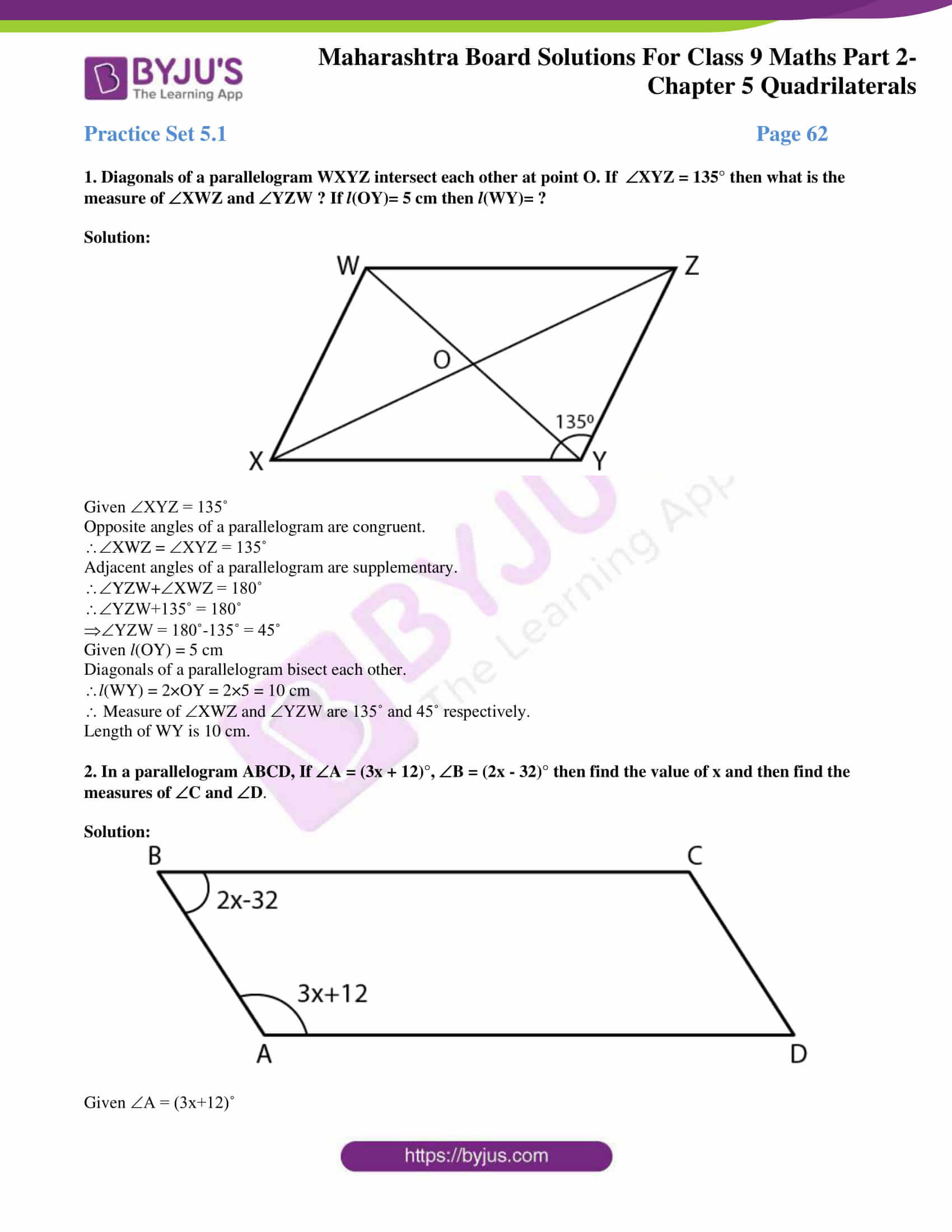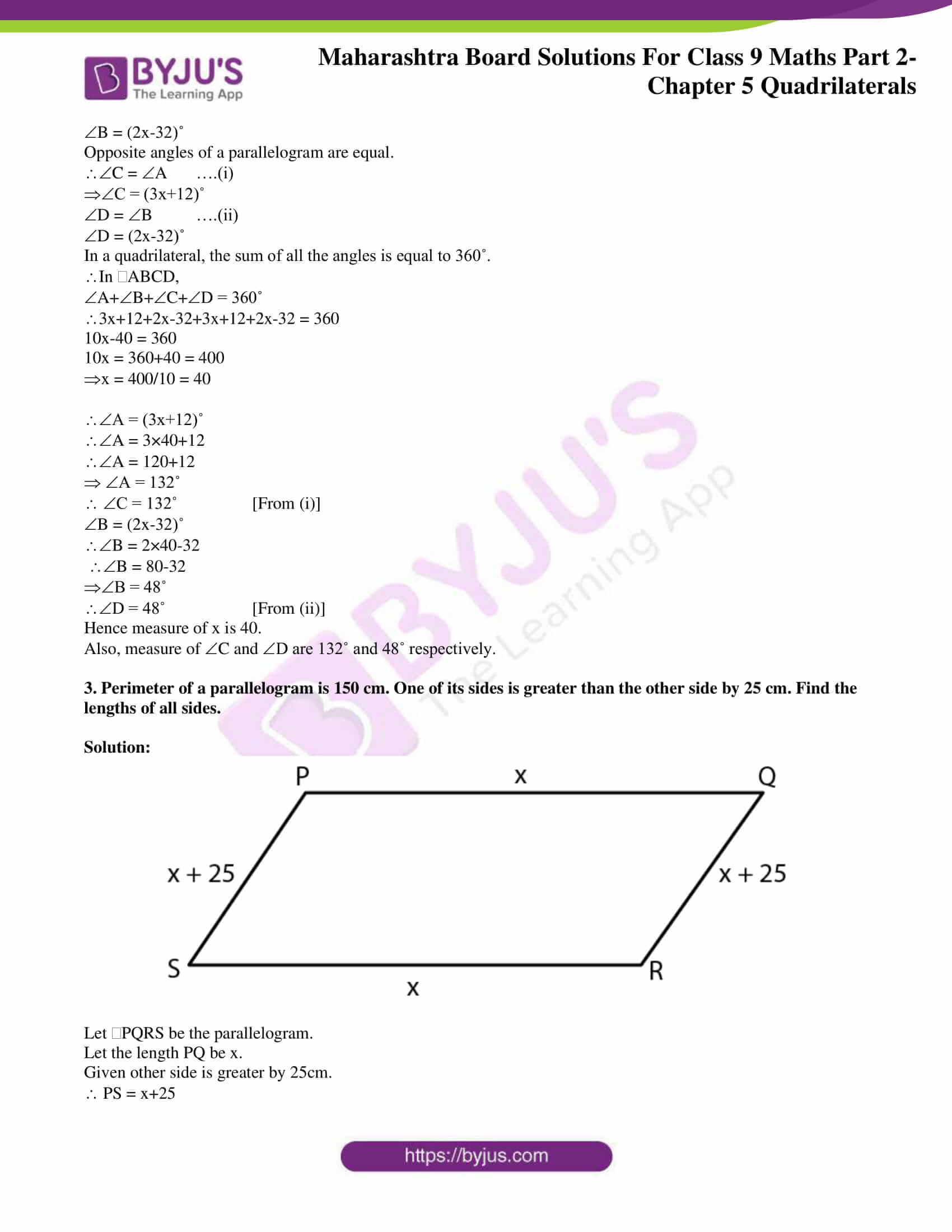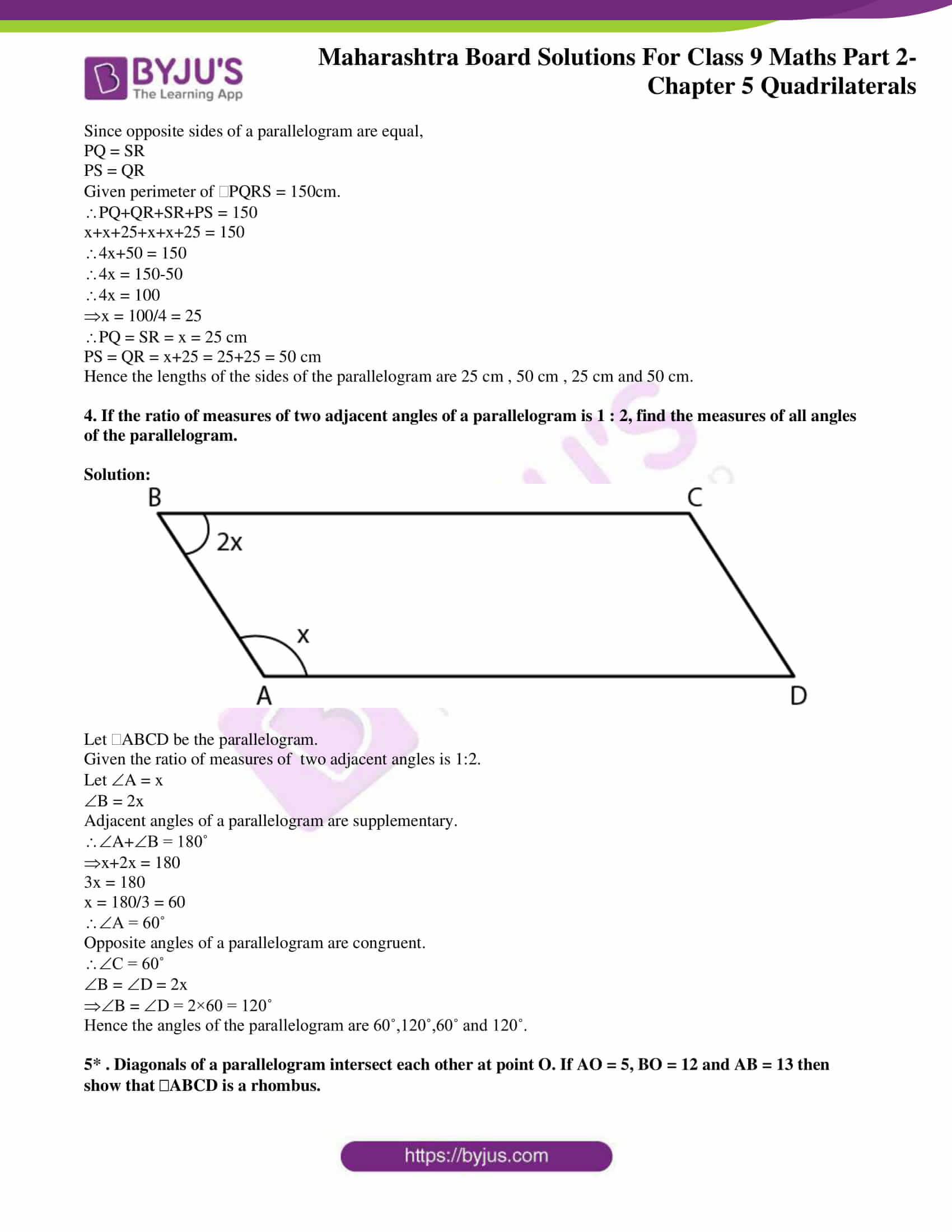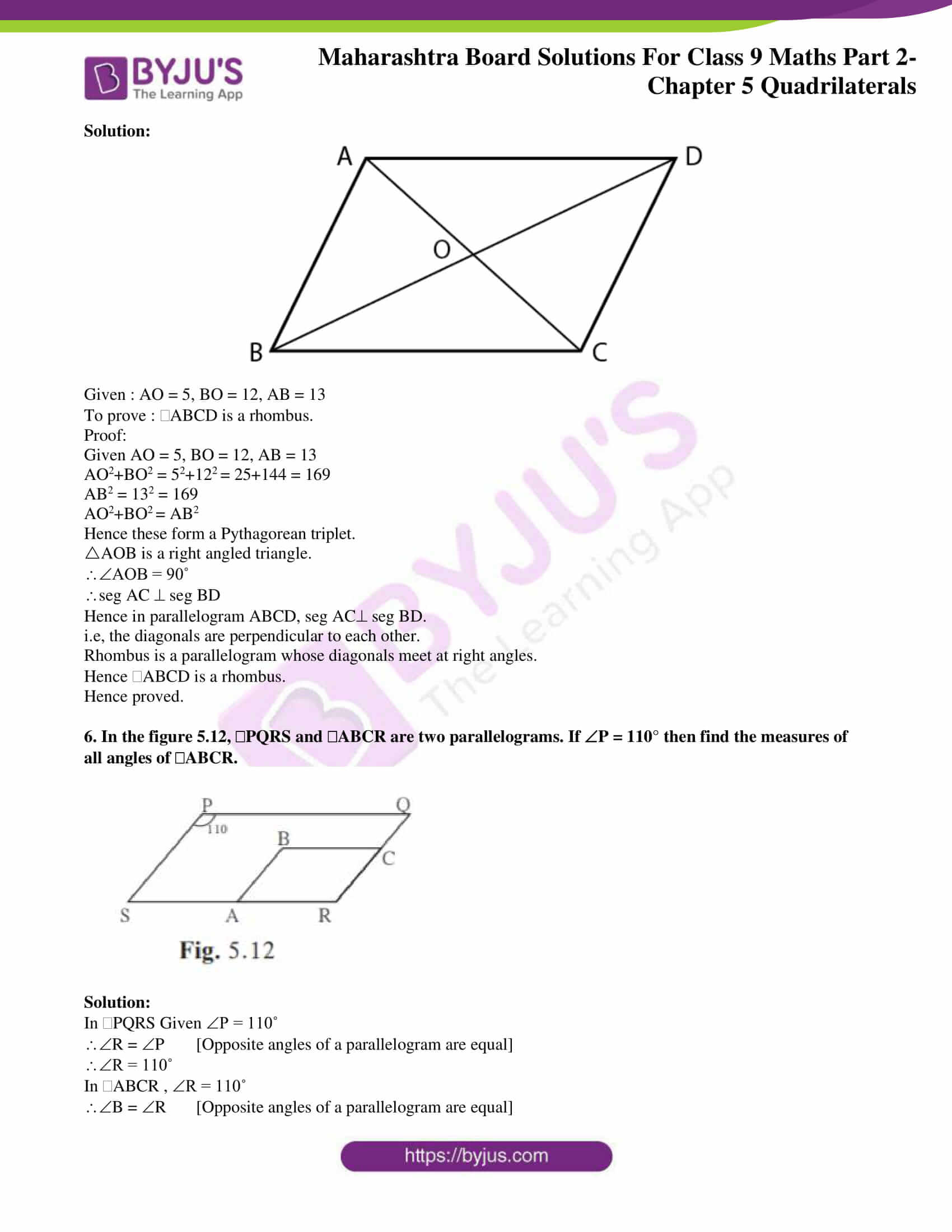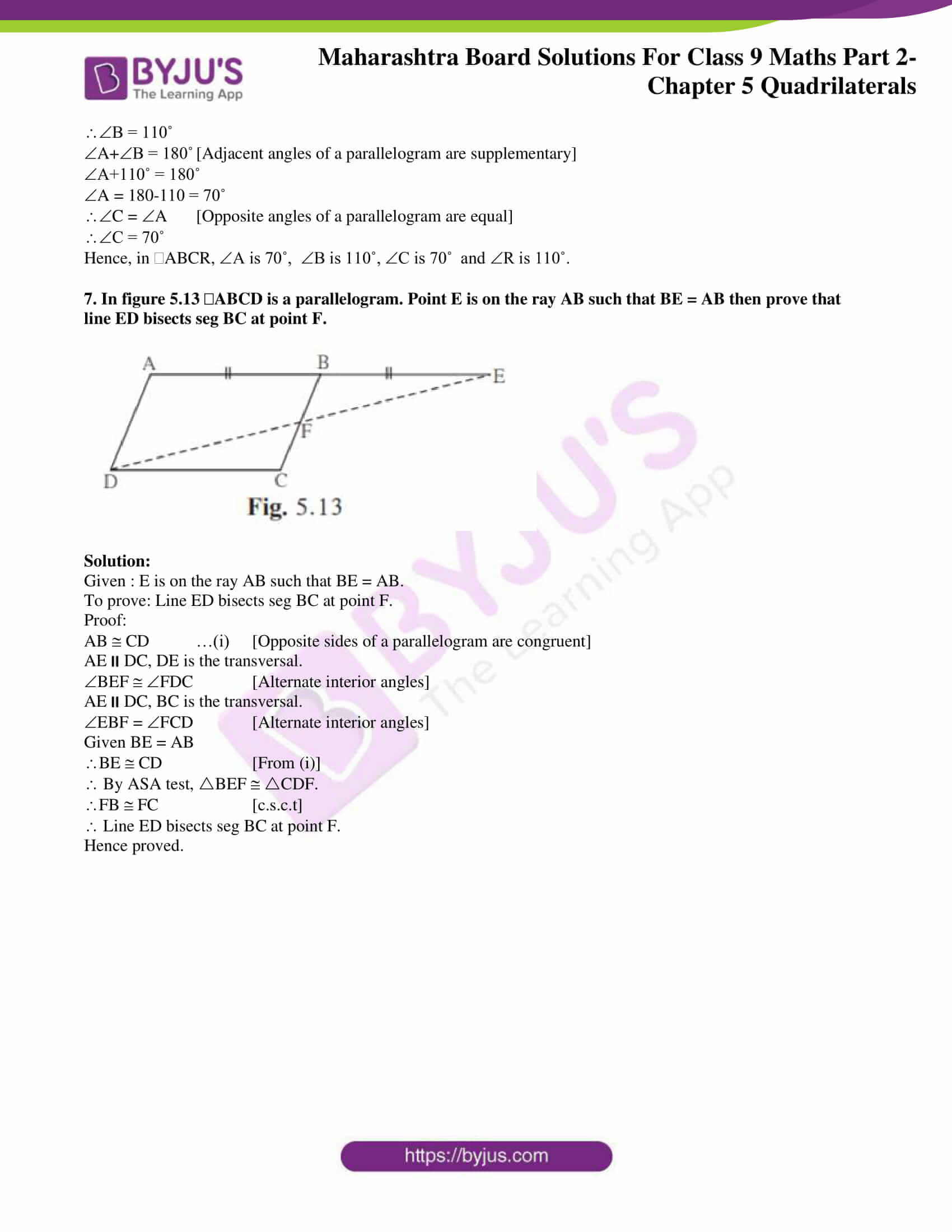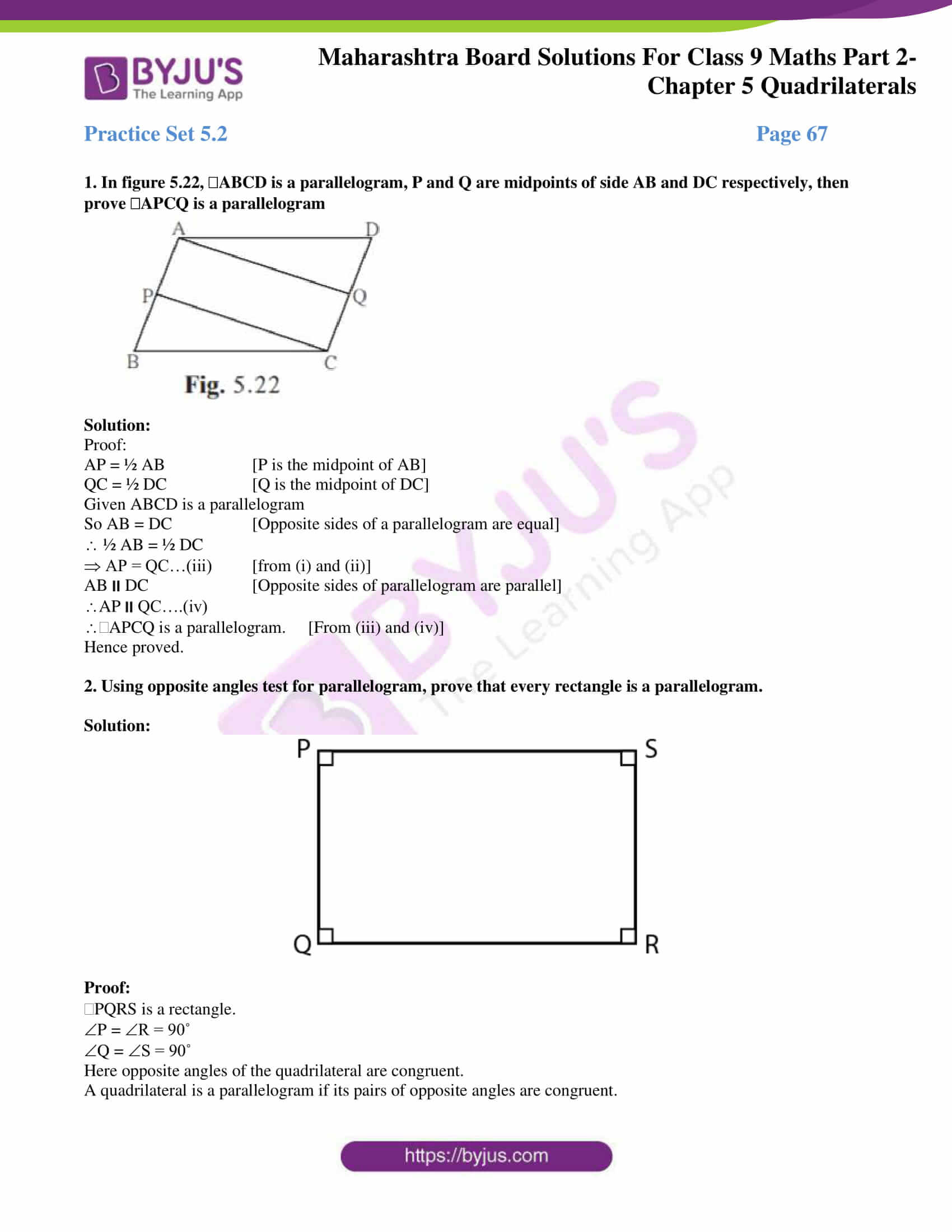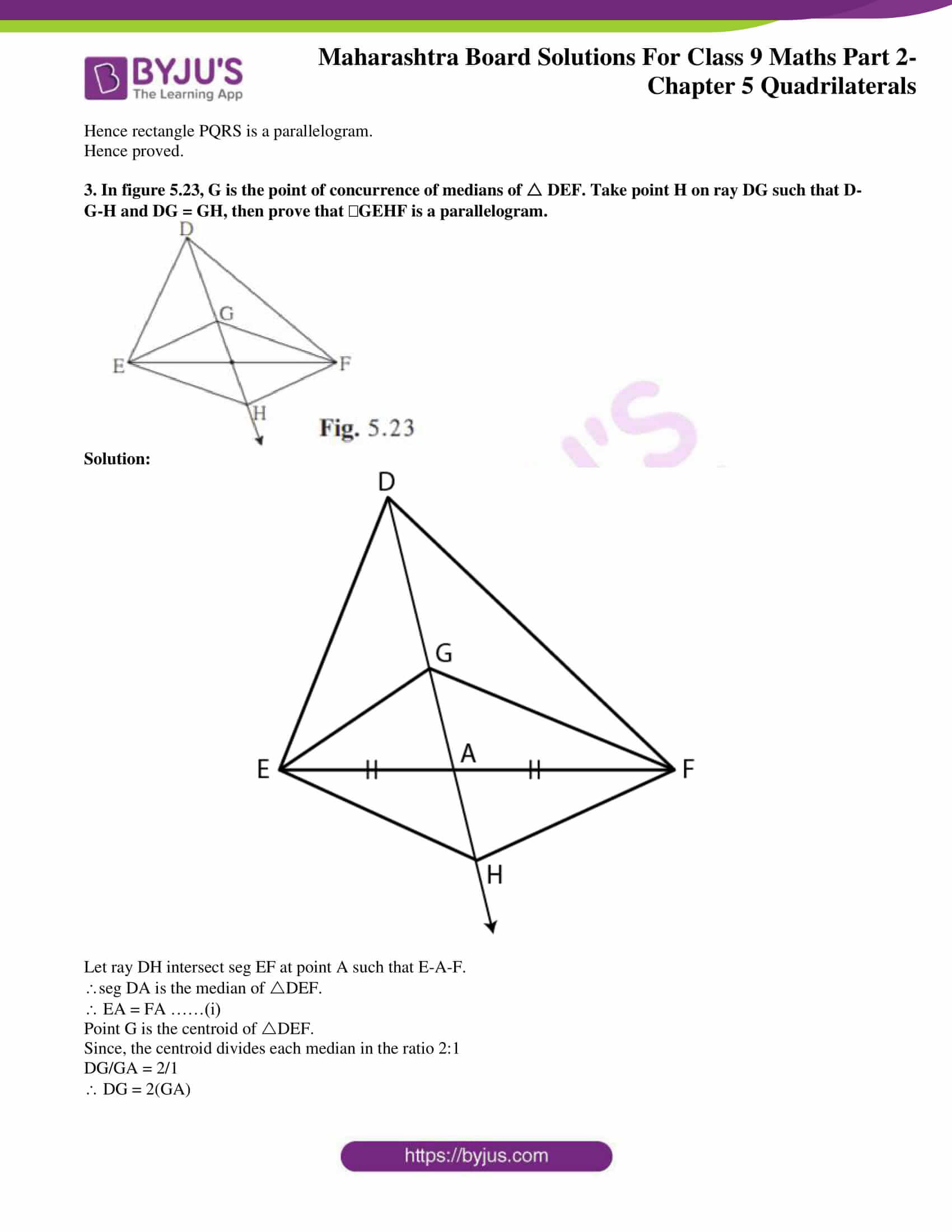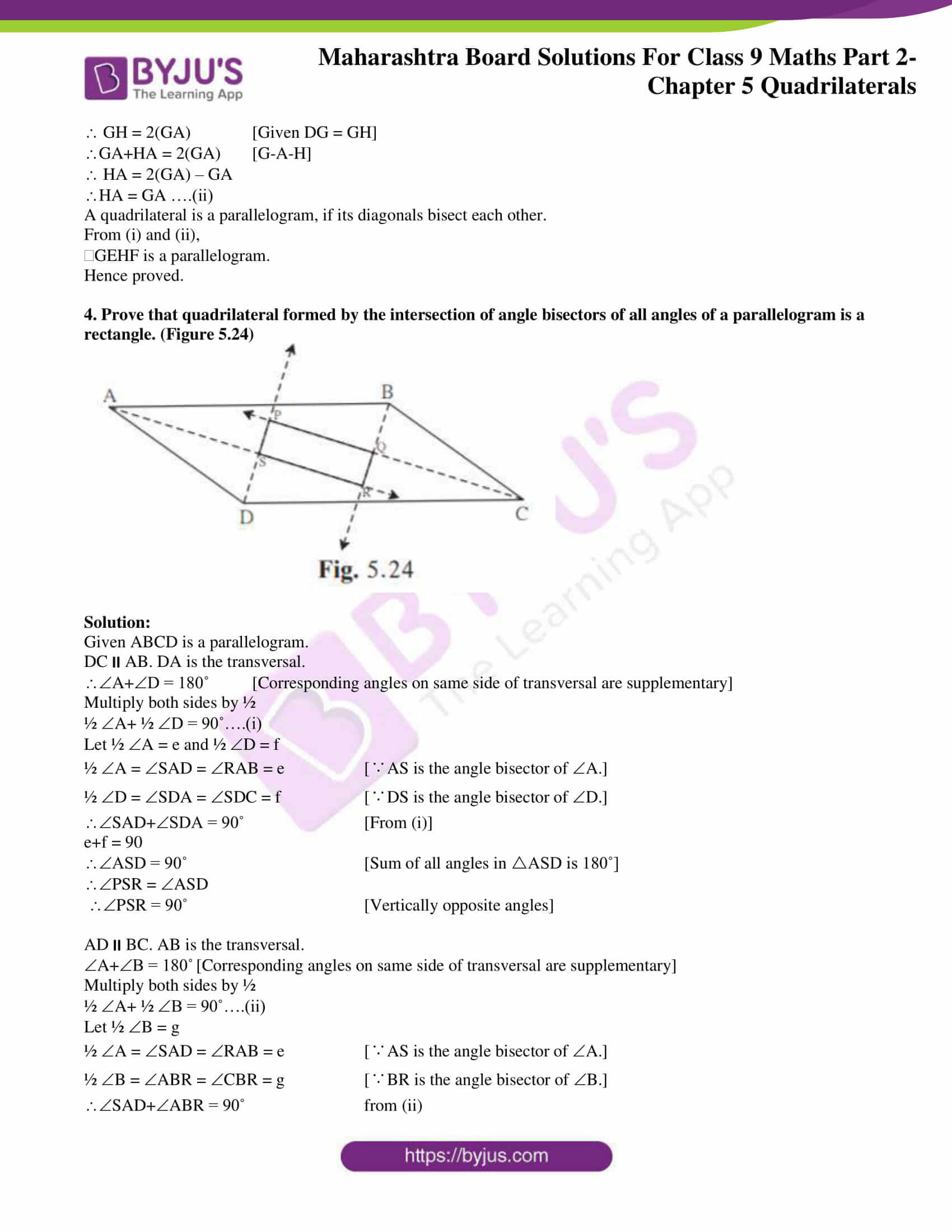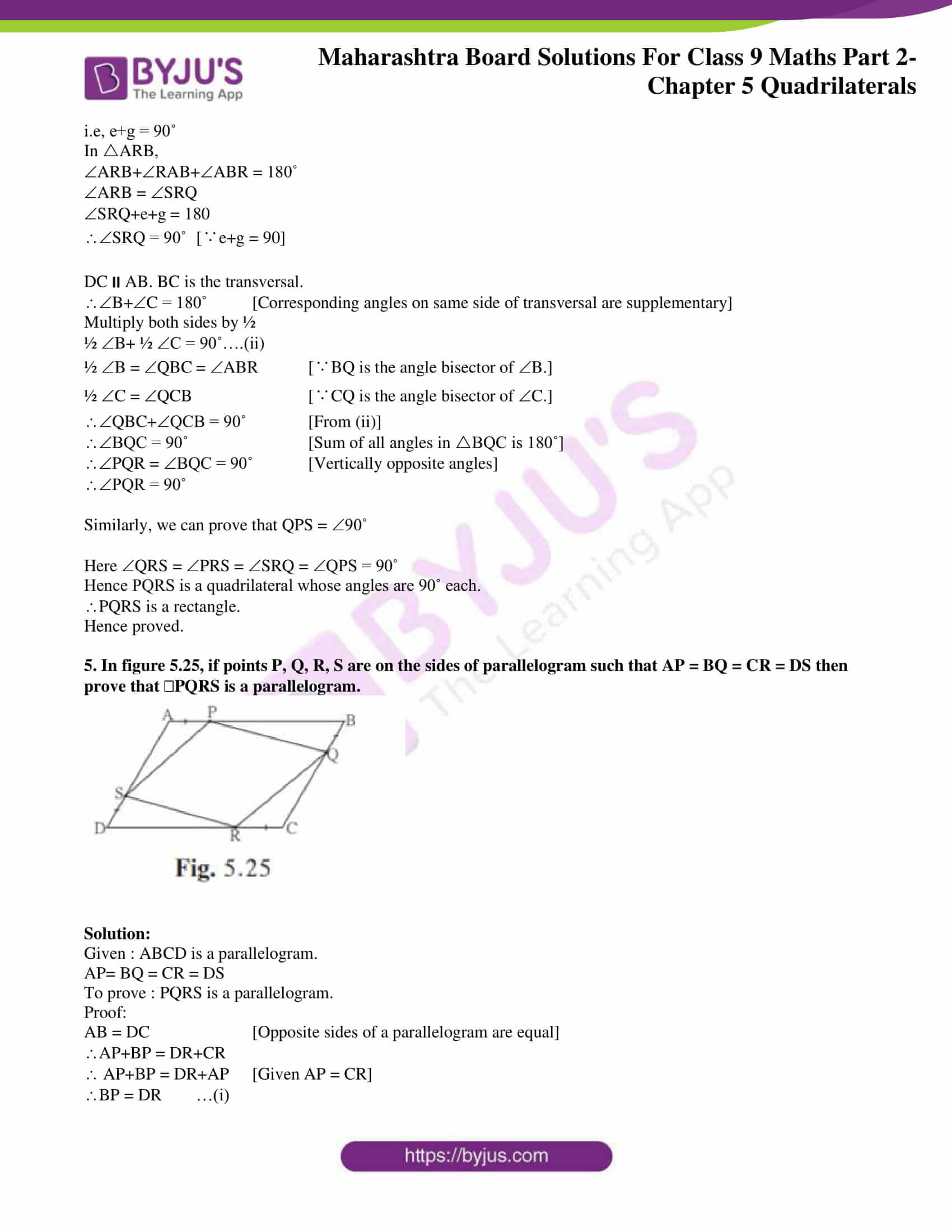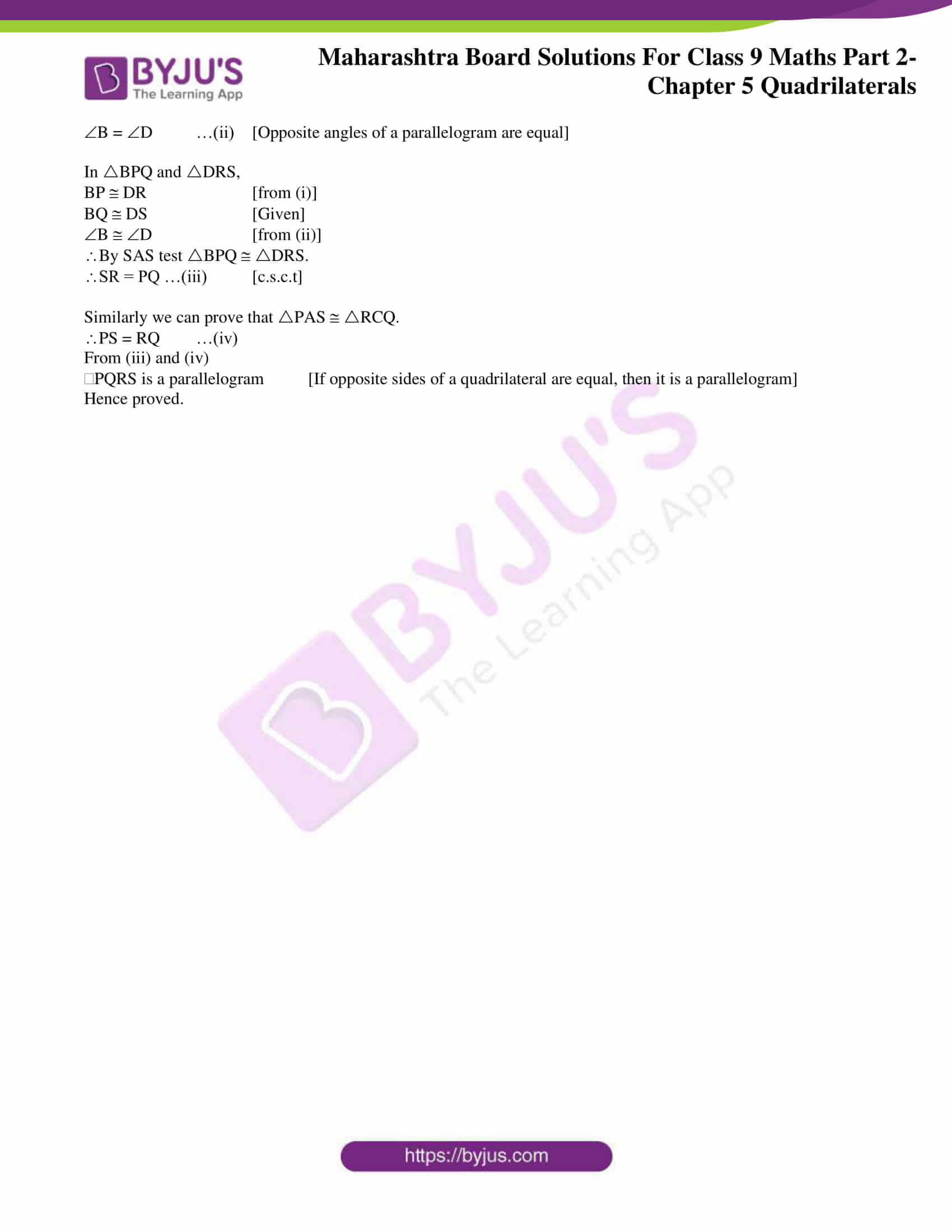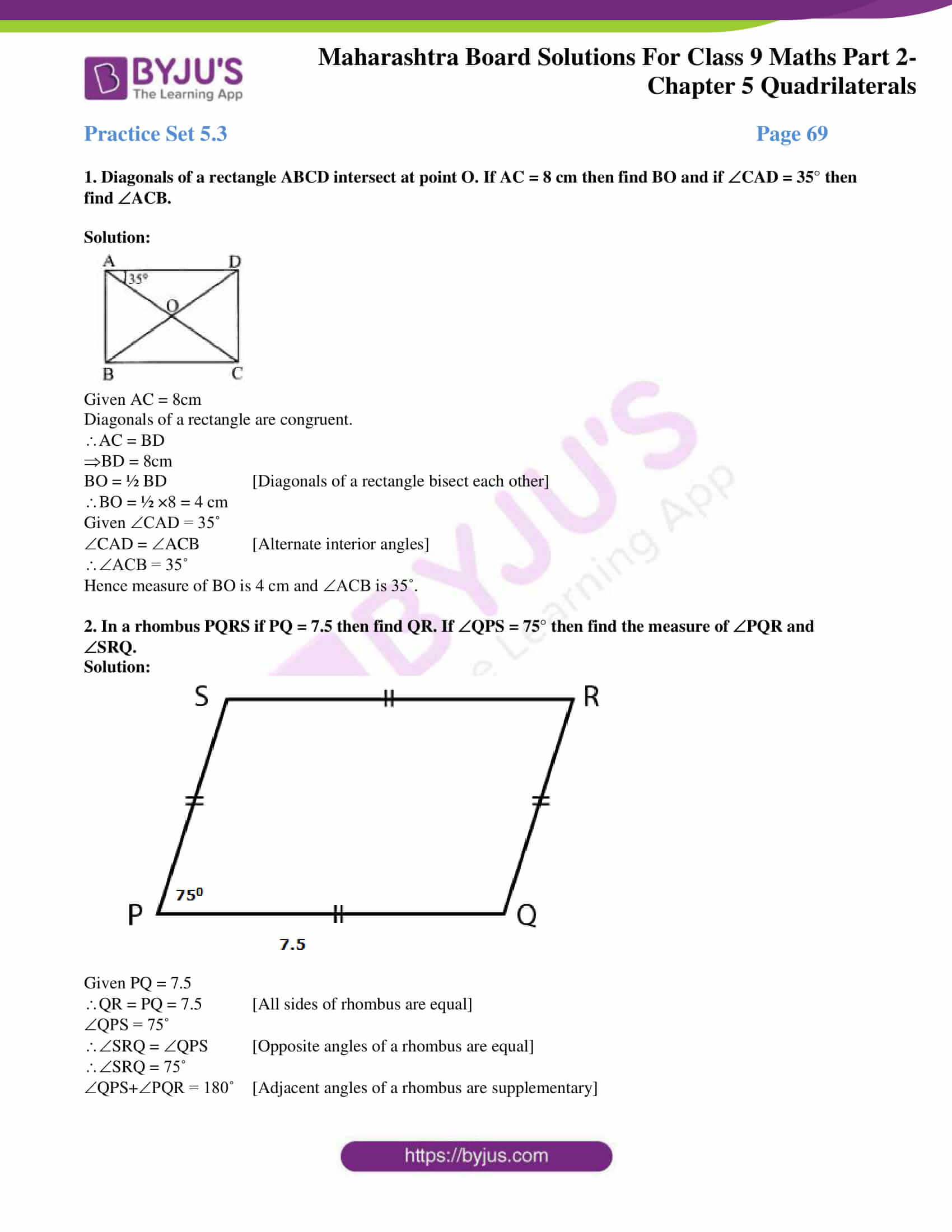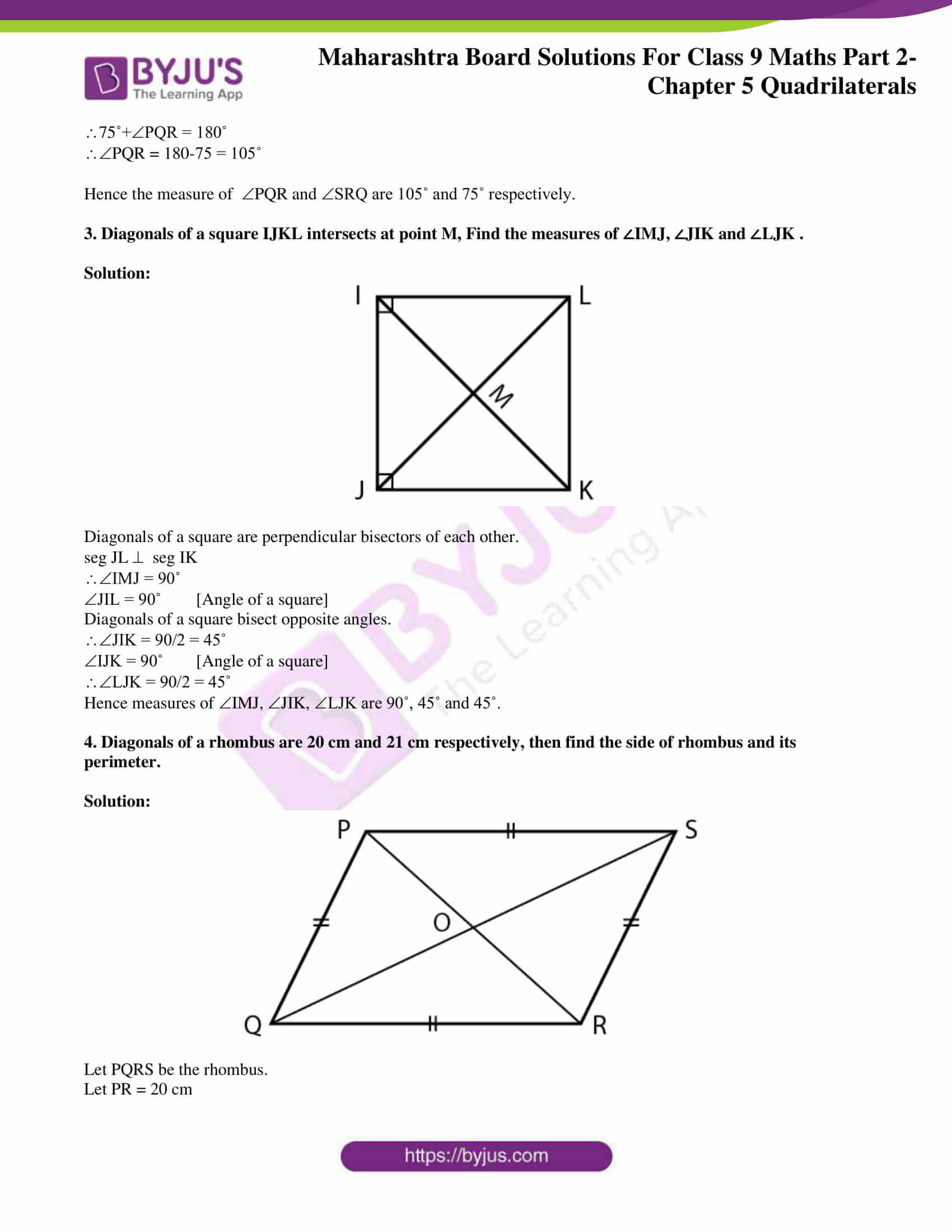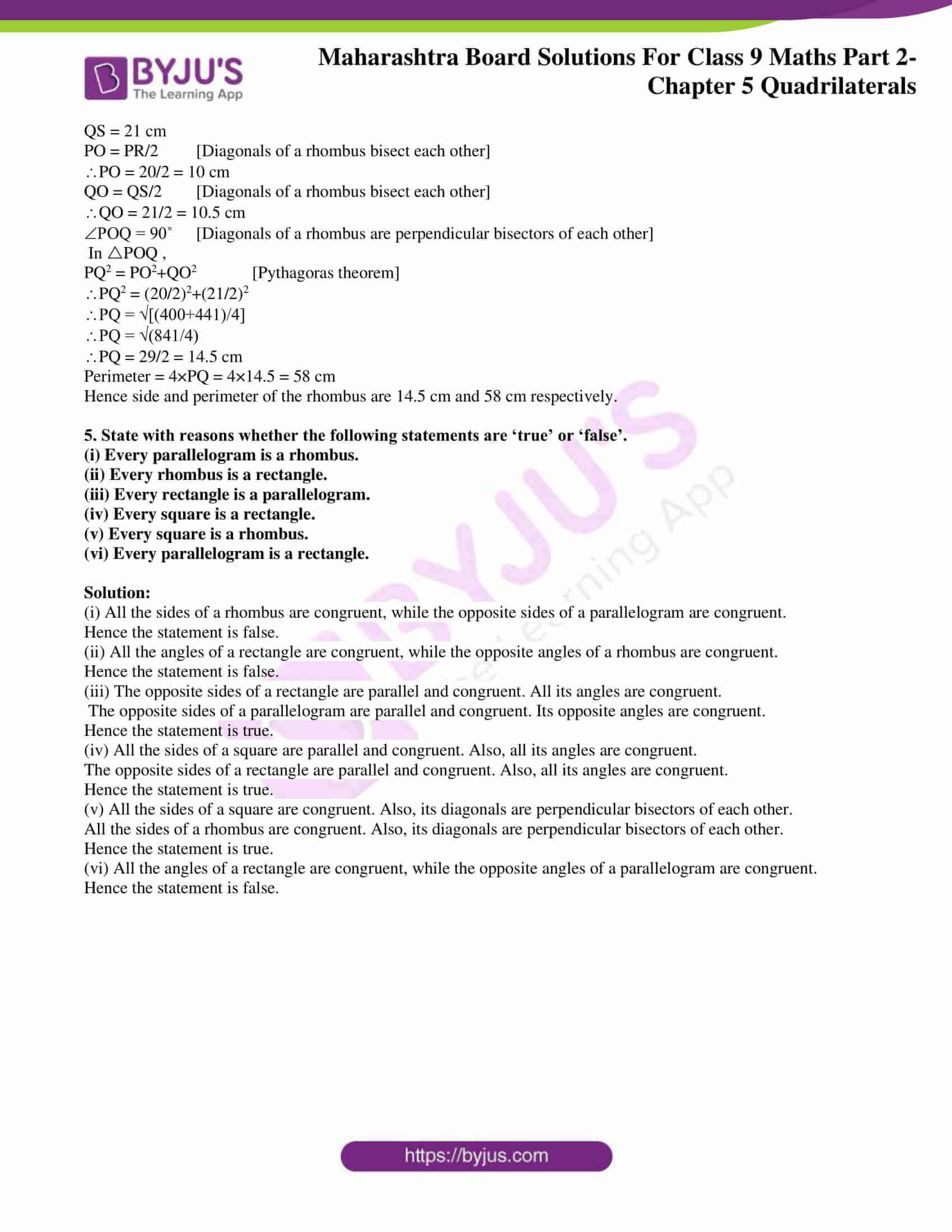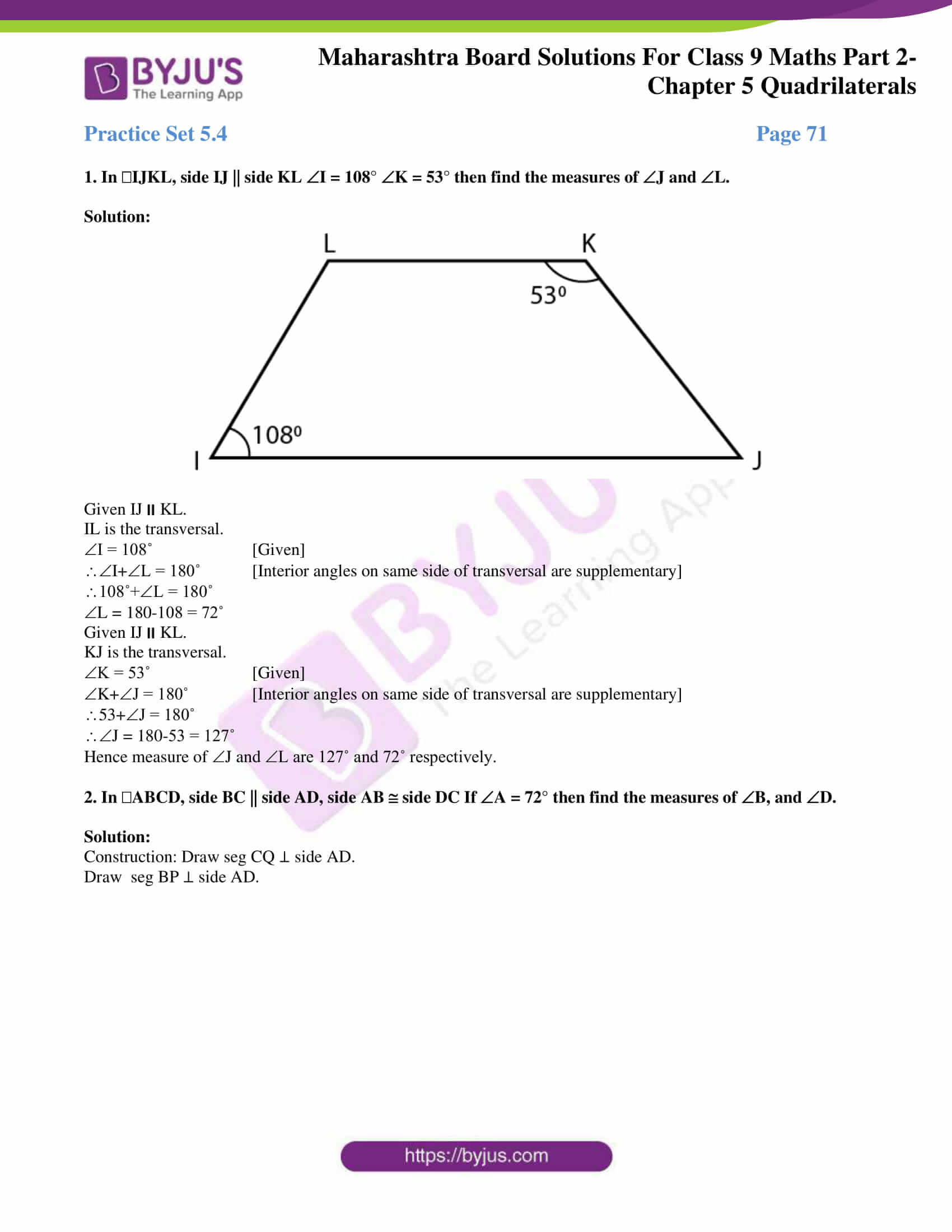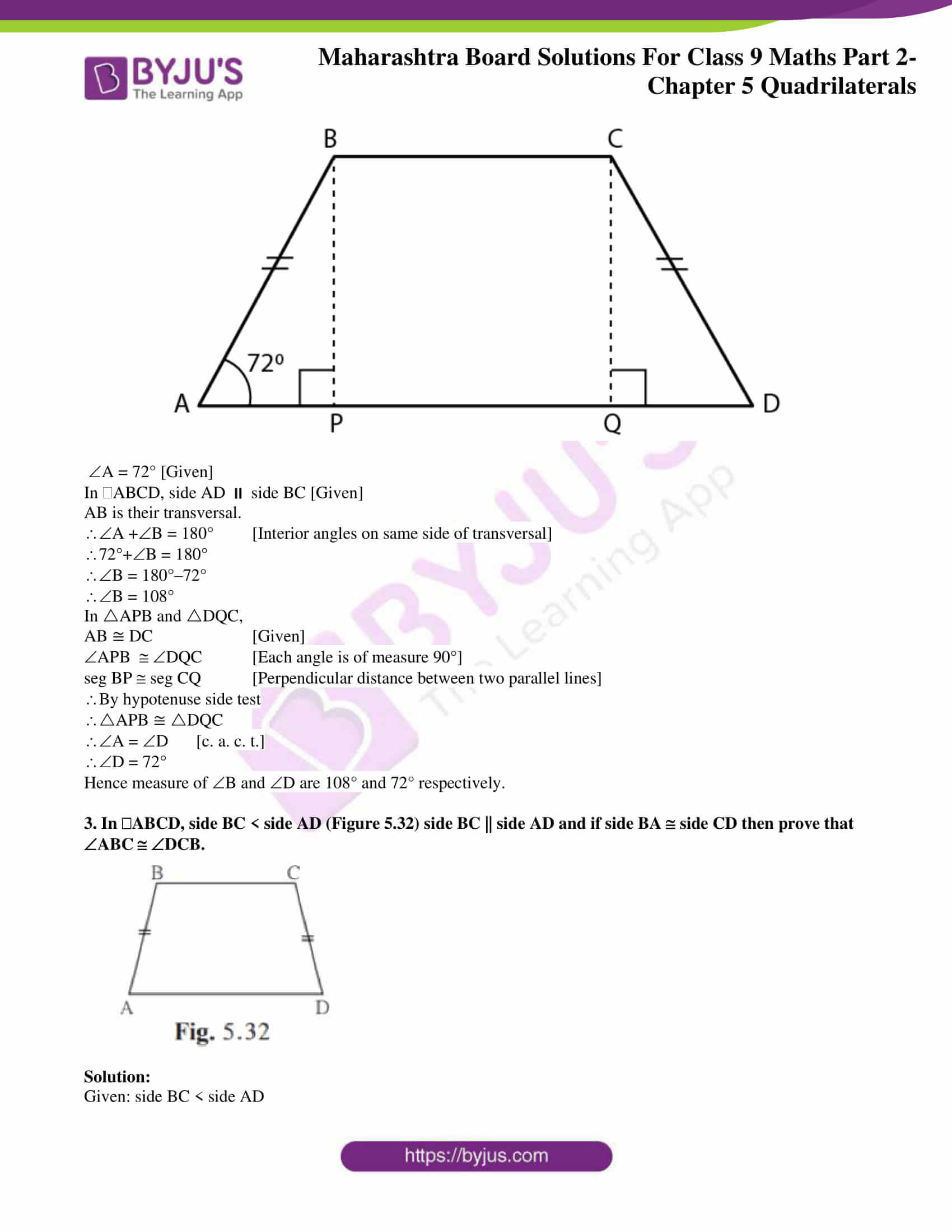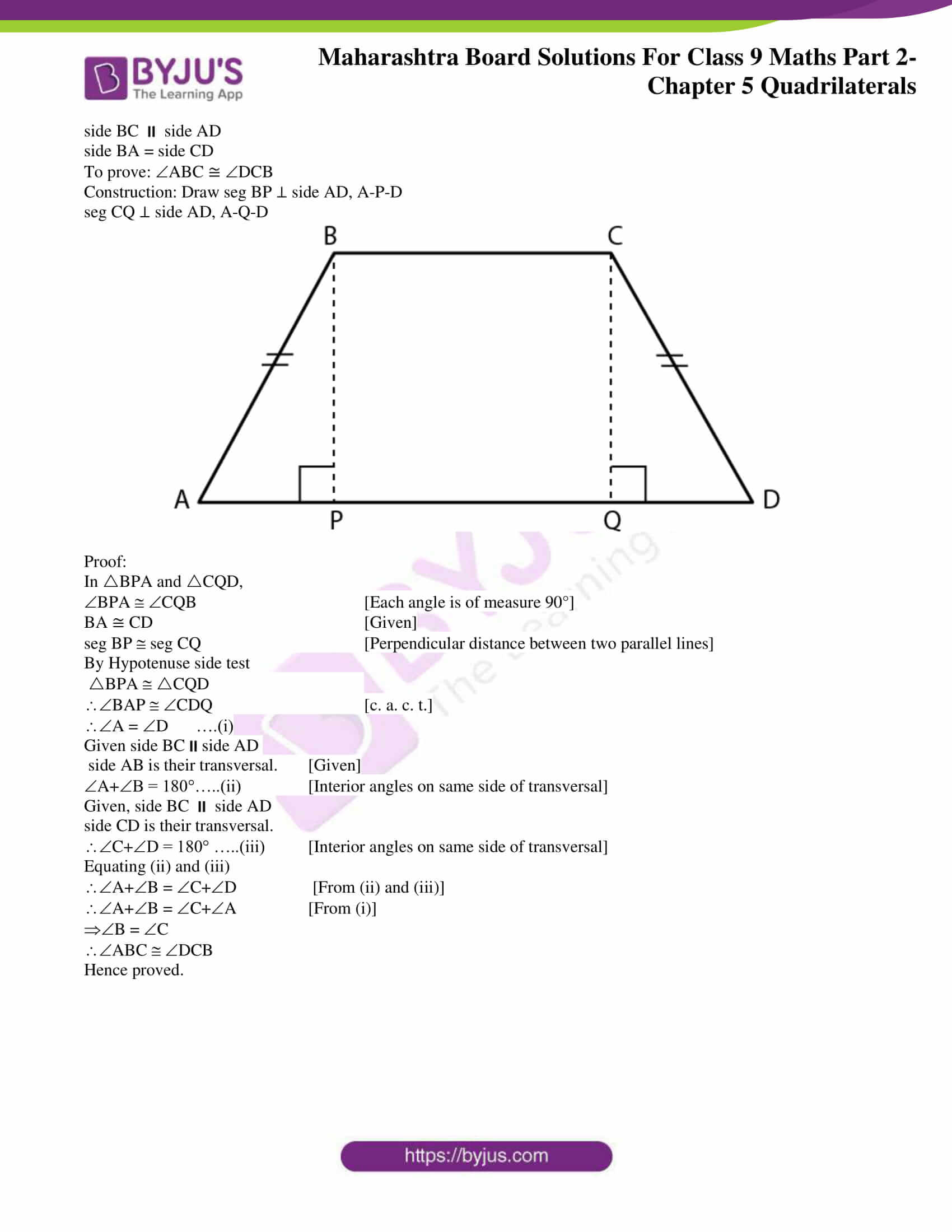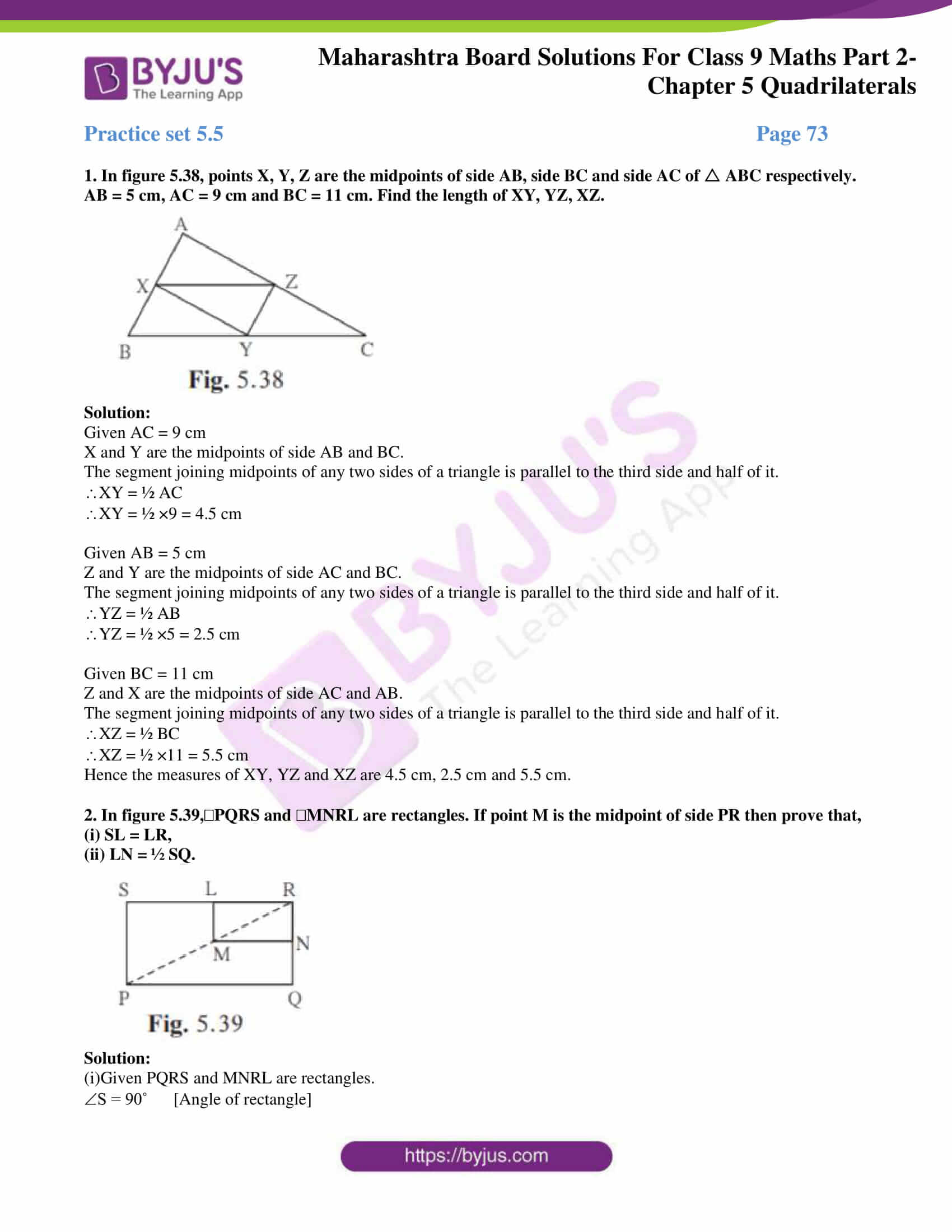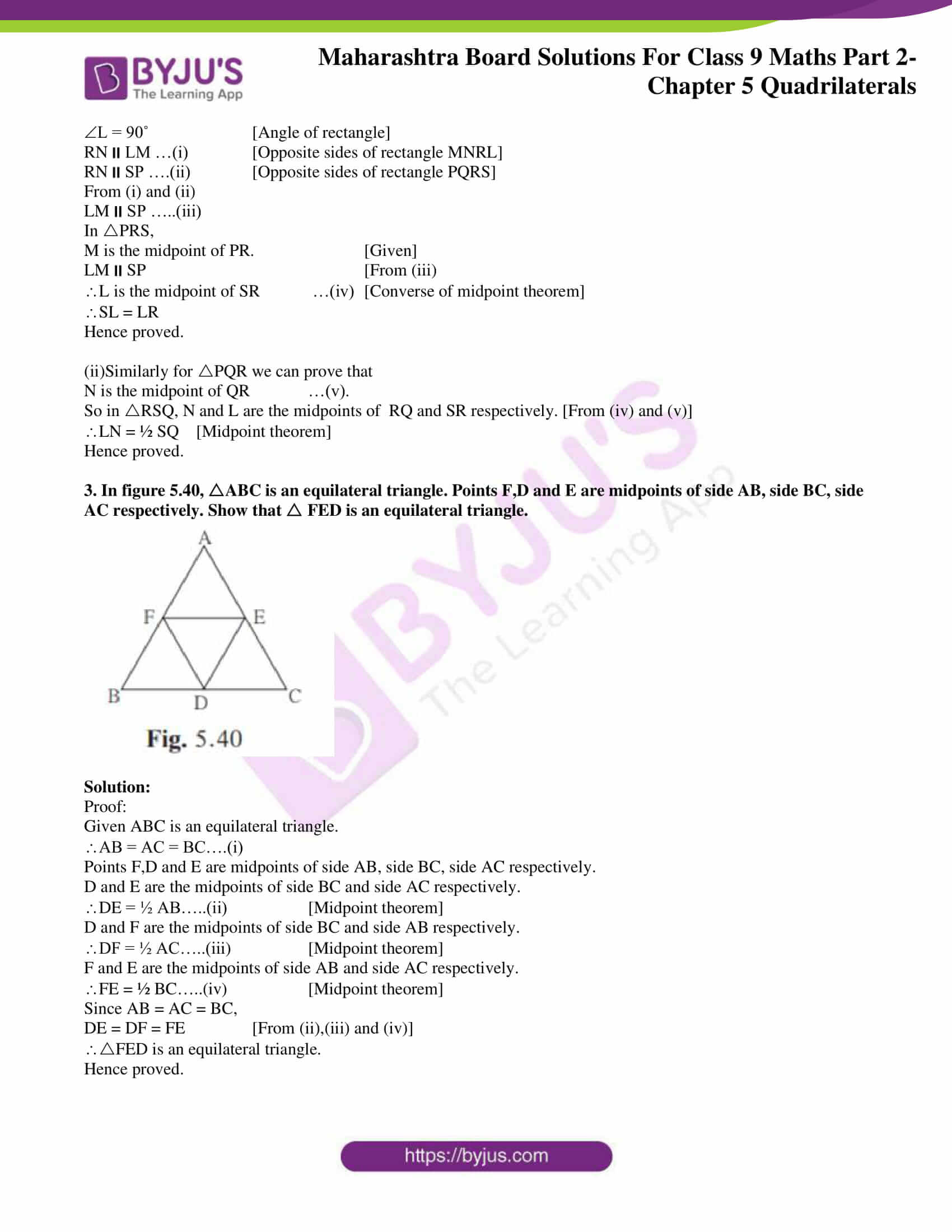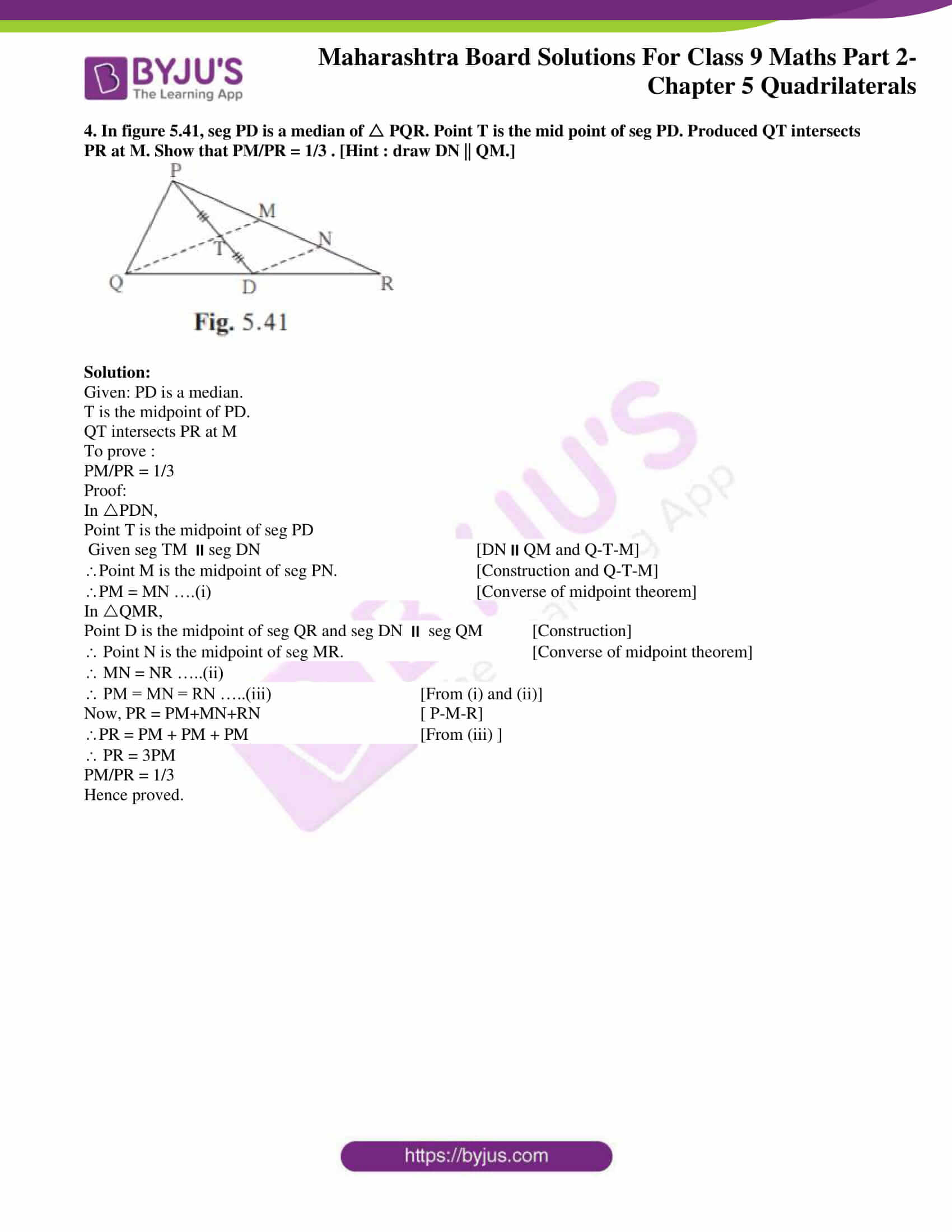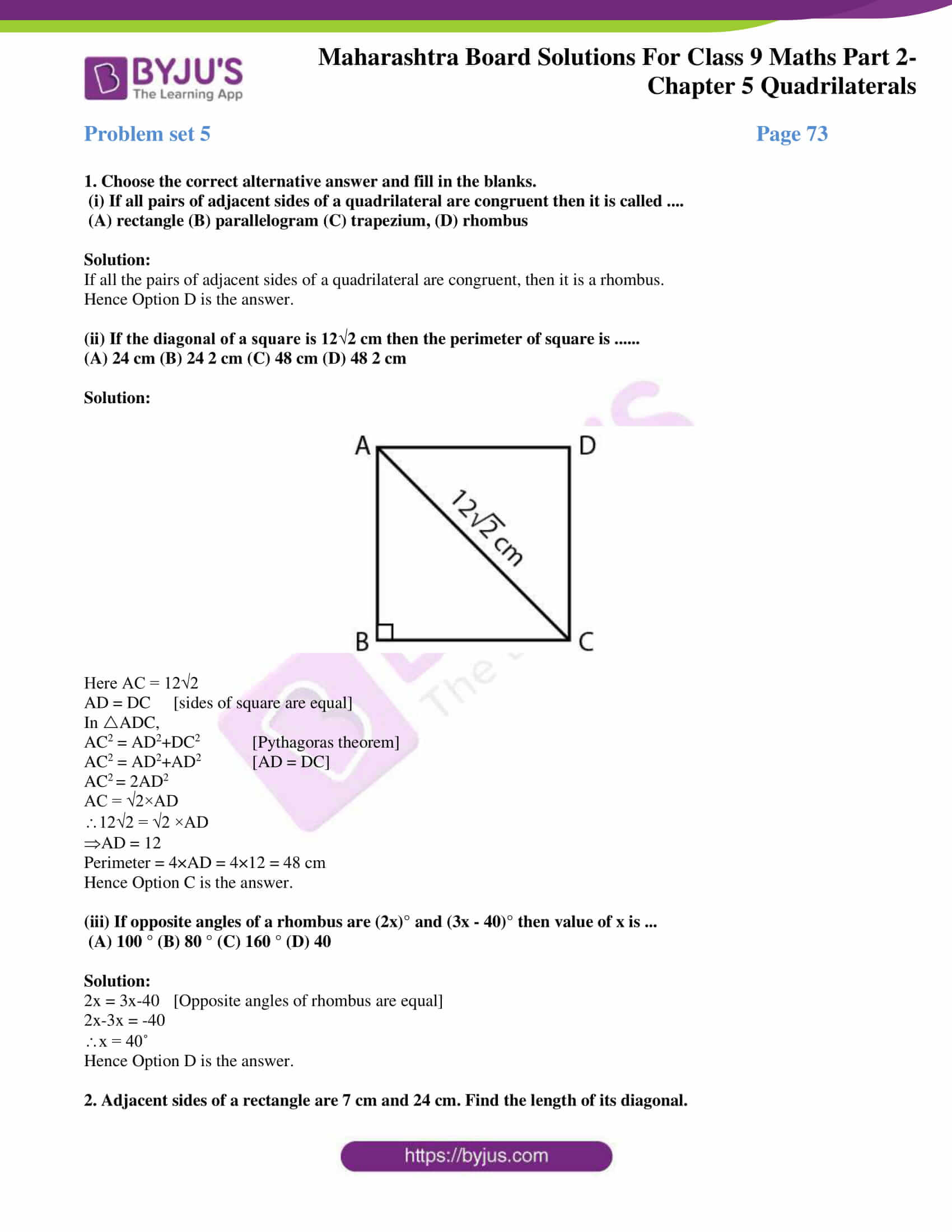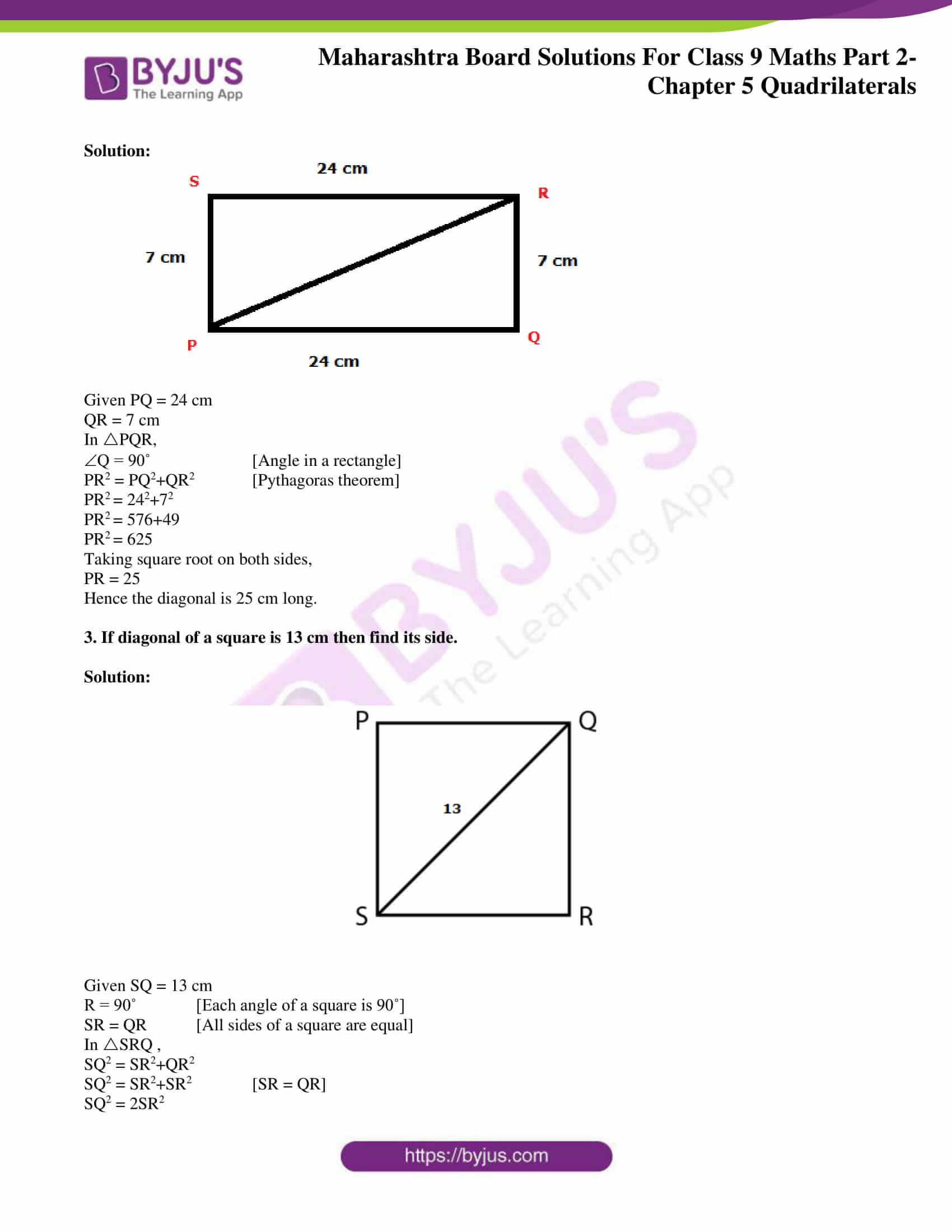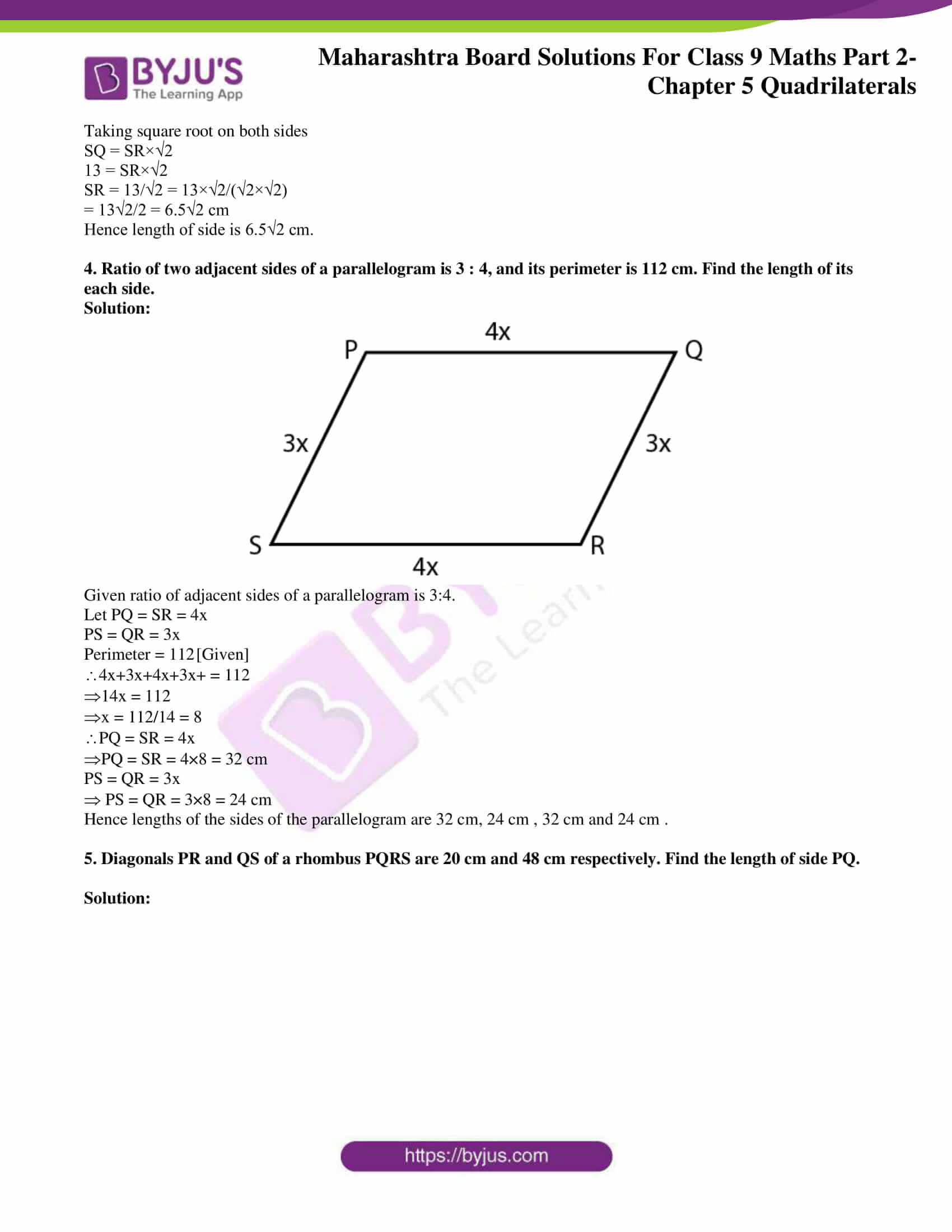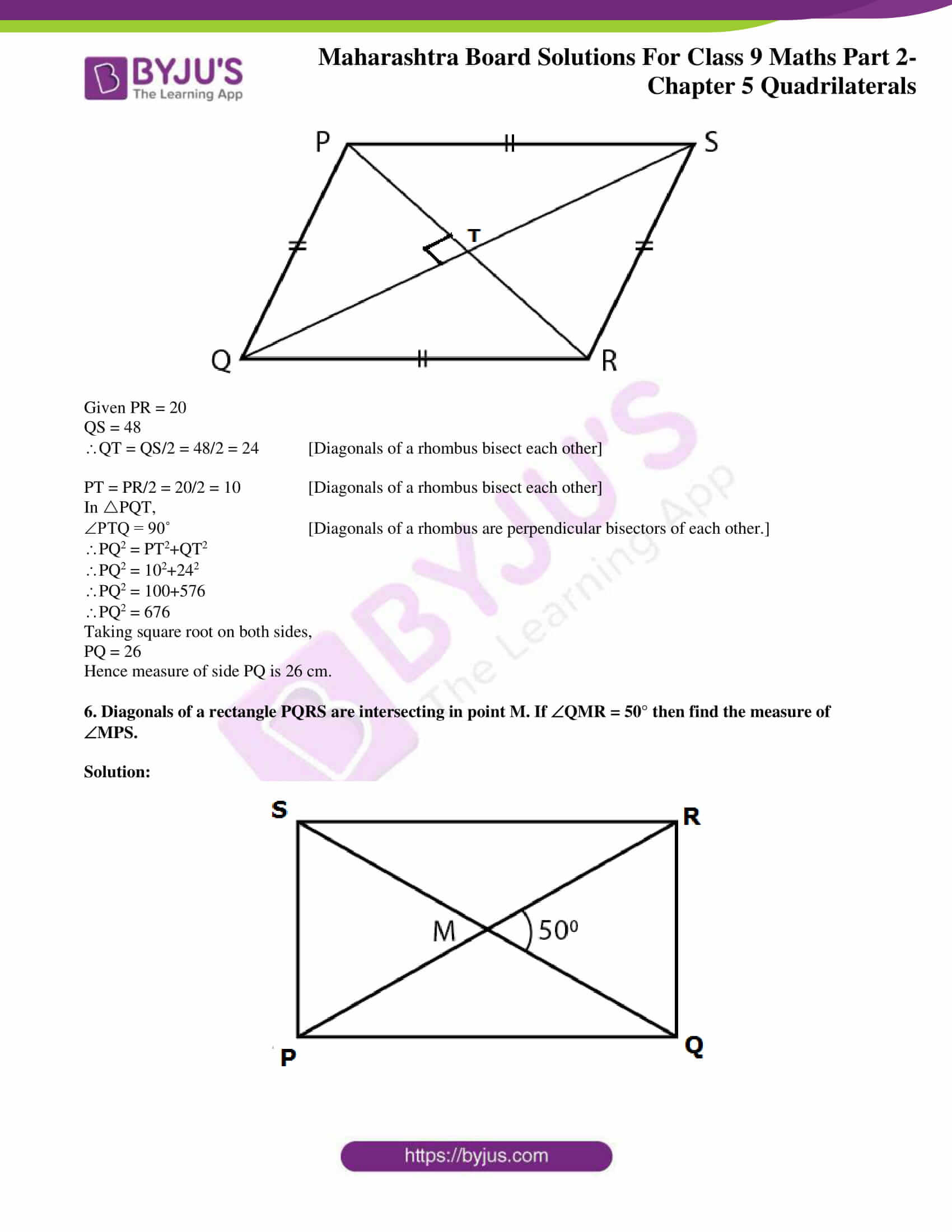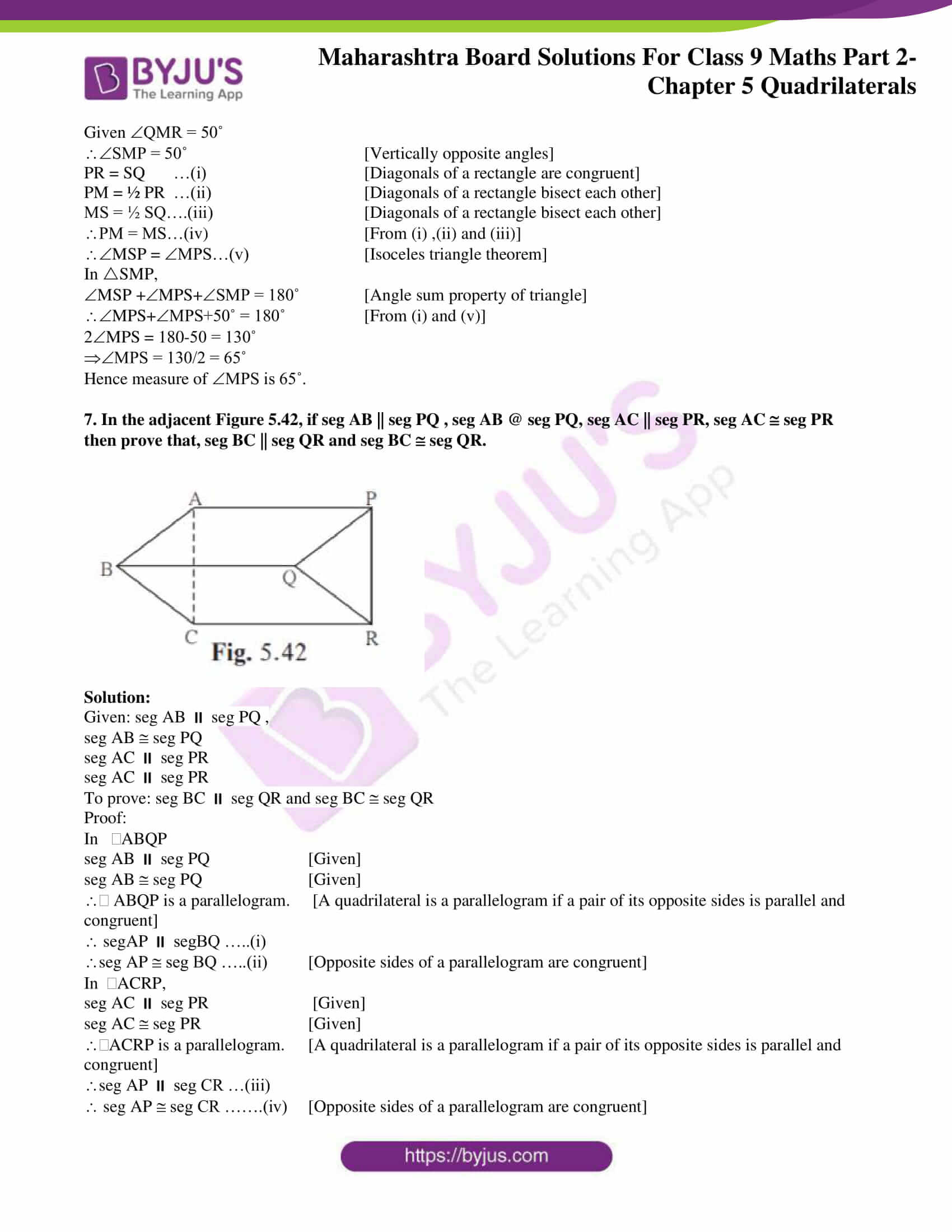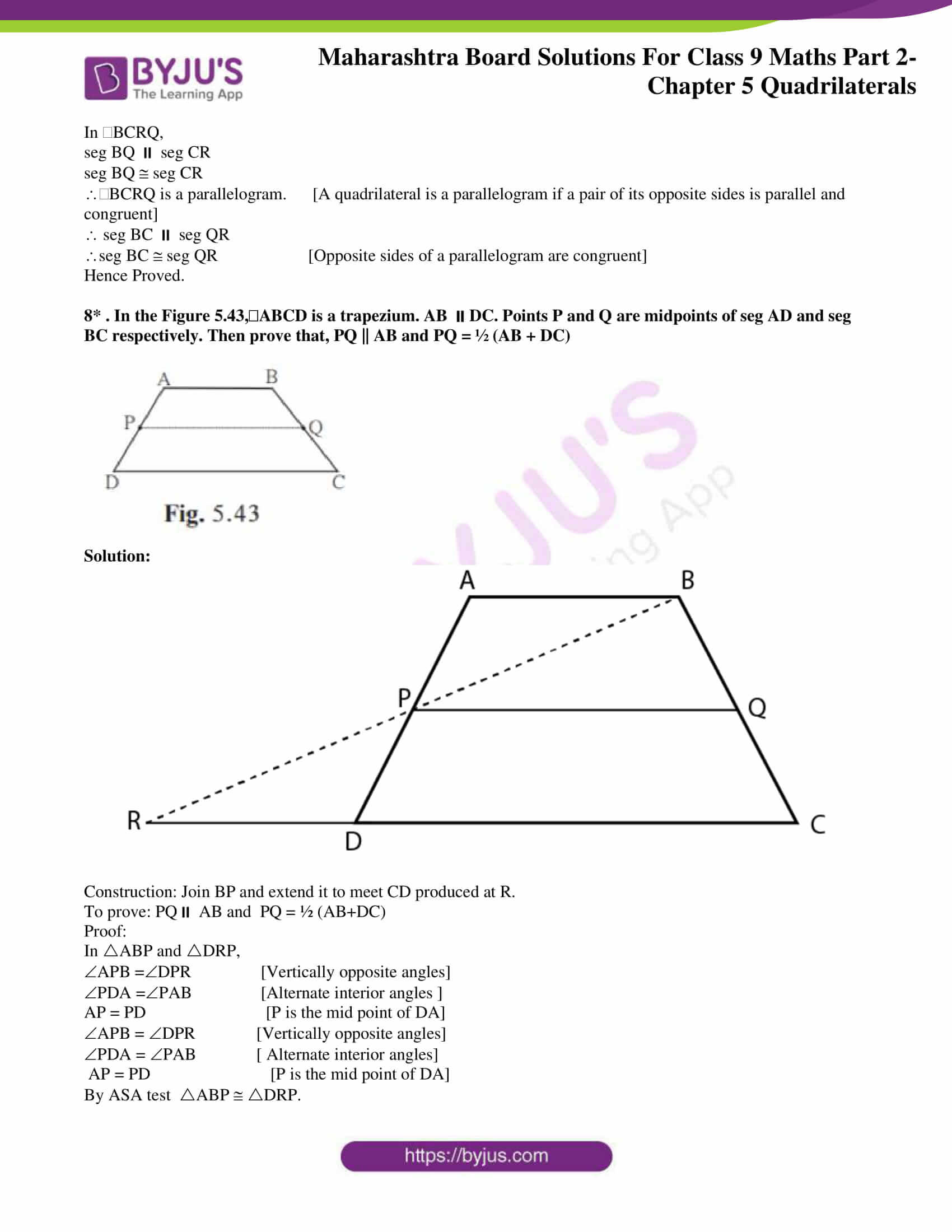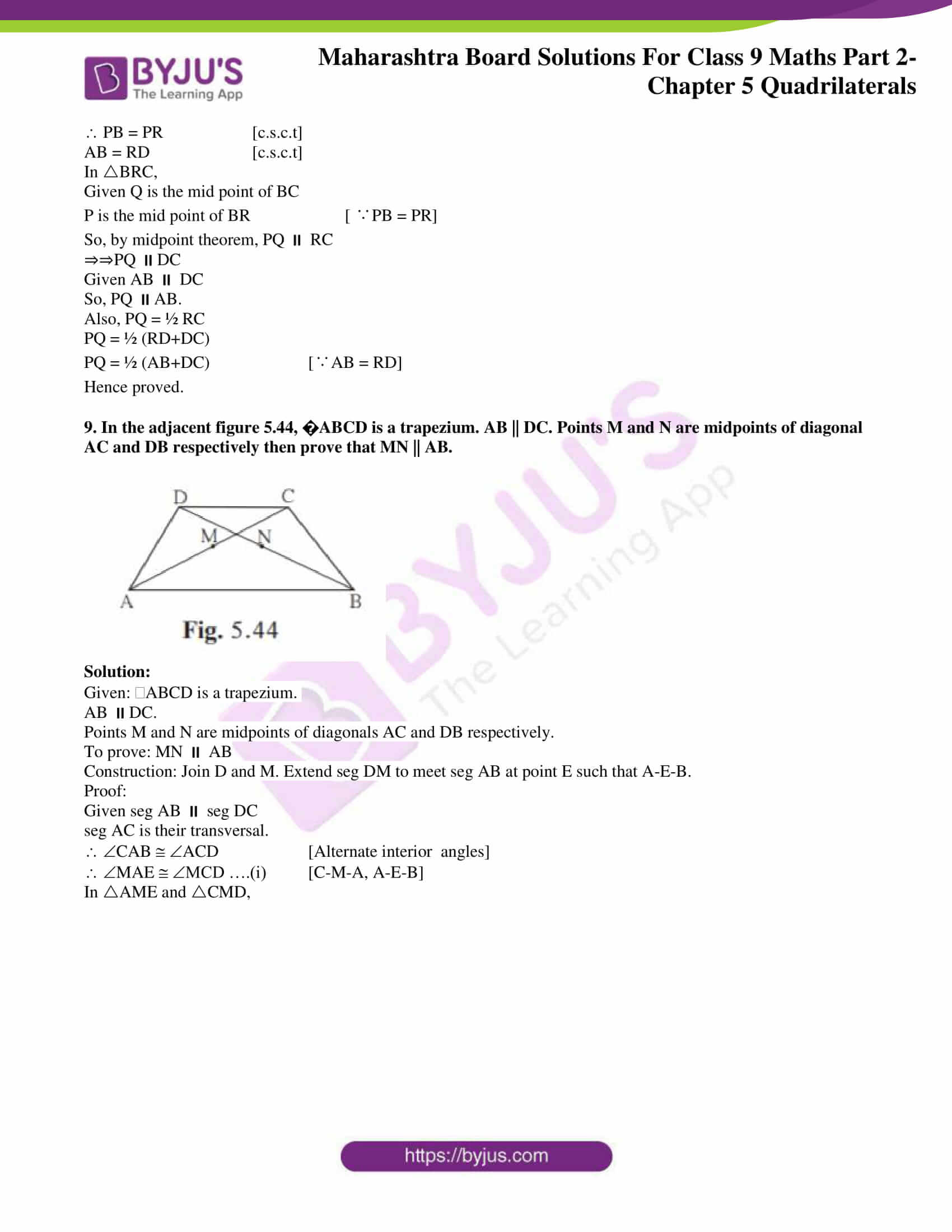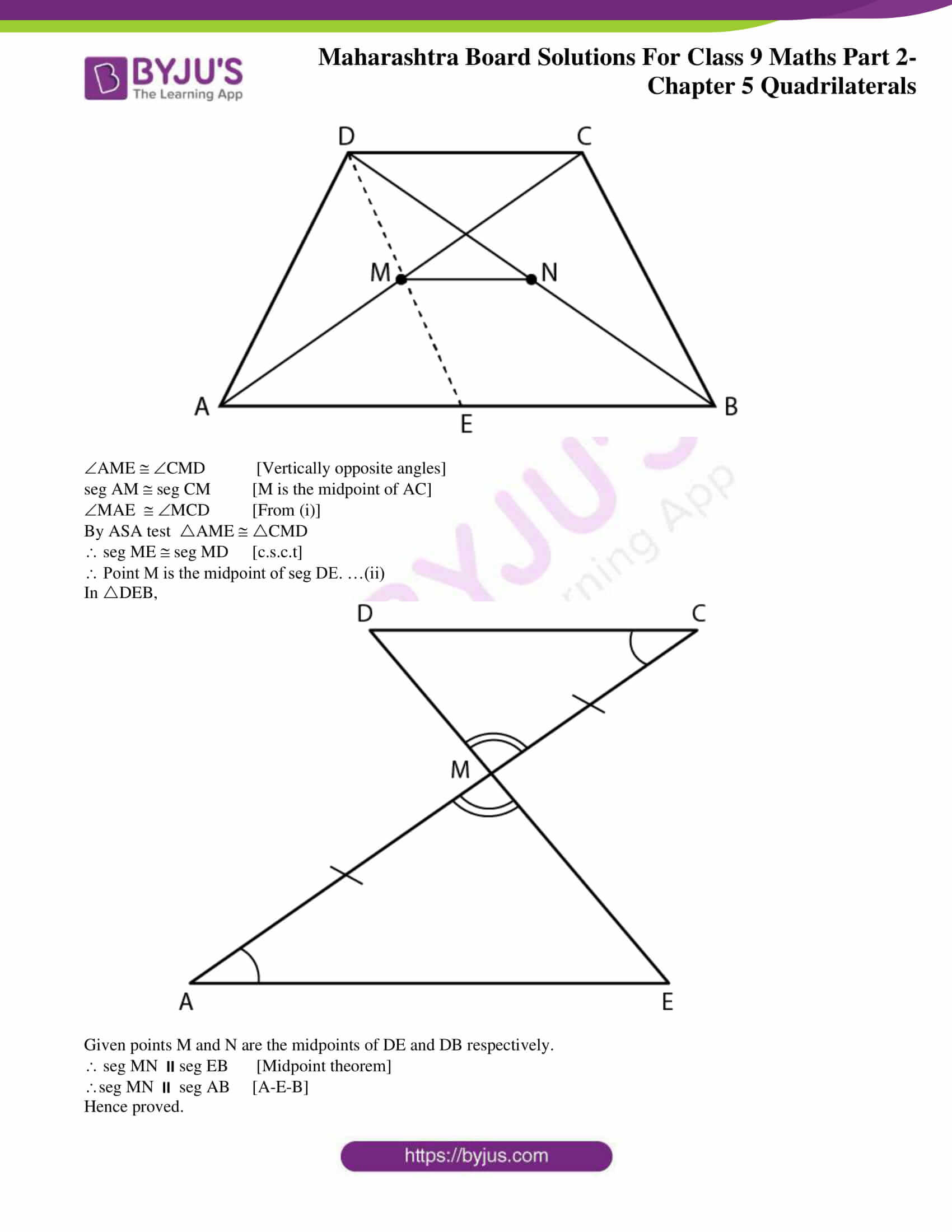### Access answers to Maths MSBSHSE Solutions For Class 9 Part 2 Chapter 5 – Quadrilaterals

Practice Set 5.1 Page 62

1. Diagonals of a parallelogram WXYZ intersect each other at point O. If XYZ = 135° then what is the measure of XWZ and YZW ? If l(OY)= 5 cm then l(WY)= ?

Solution: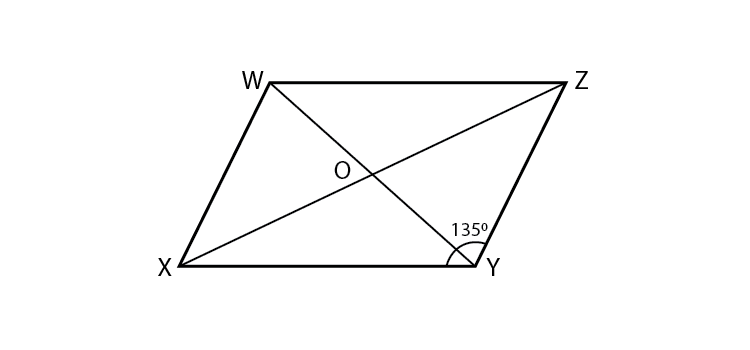Given XYZ = 135˚

Opposite angles of a parallelogram are congruent.

XWZ = XYZ = 135˚

Adjacent angles of a parallelogram are supplementary.

YZW+XWZ = 180˚

YZW+135˚ = 180˚

YZW = 180˚-135˚ = 45˚

Given l(OY) = 5 cm

Diagonals of a parallelogram bisect each other.

l(WY) = 2×OY = 2×5 = 10 cm

Measure of XWZ and YZW are 135˚ and 45˚ respectively.

Length of WY is 10 cm.

2. In a parallelogram ABCD, If A = (3x + 12)°, B = (2x – 32)° then find the value of x and then find the measures of C and D.

Solution: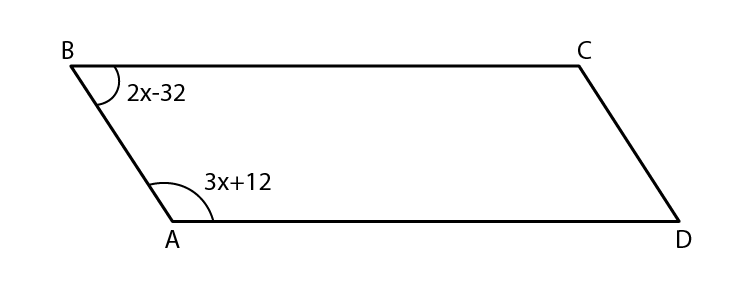Given A = (3x+12)˚

B = (2x-32)˚

Opposite angles of a parallelogram are equal.

C = A ….(i)

C = (3x+12)˚

D = B ….(ii)

D = (2x-32)˚

In a quadrilateral, the sum of all the angles is equal to 360˚.

In ABCD,

A+B+C+D = 360˚

3x+12+2x-32+3x+12+2x-32 = 360

10x-40 = 360

10x = 360+40 = 400

x = 400/10 = 40

A = (3x+12)˚

A = 3×40+12

A = 120+12

A = 132˚

C = 132˚ [From (i)]

B = (2x-32)˚

B = 2×40-32

B = 80-32

B = 48˚

D = 48˚ [From (ii)]

Hence measure of x is 40.

Also, measure of C and D are 132˚ and 48˚ respectively.

3. Perimeter of a parallelogram is 150 cm. One of its sides is greater than the other side by 25 cm. Find the lengths of all sides.

Solution: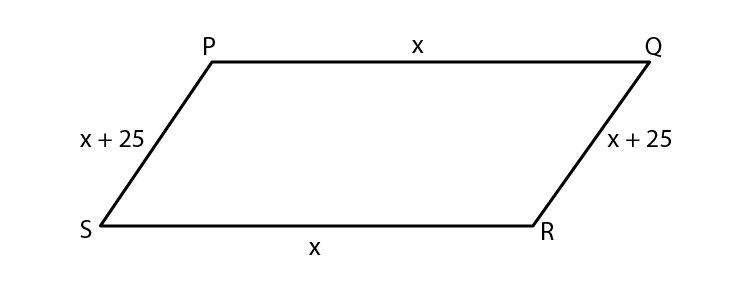Let PQRS be the parallelogram.

Let the length PQ be x.

Given other side is greater by 25cm.

PS = x+25

Since opposite sides of a parallelogram are equal,

PQ = SR

PS = QR

Given perimeter of PQRS = 150cm.

PQ+QR+SR+PS = 150

x+x+25+x+x+25 = 150

4x+50 = 150

4x = 150-50

4x = 100

x = 100/4 = 25

PQ = SR = x = 25 cm

PS = QR = x+25 = 25+25 = 50 cm

Hence the lengths of the sides of the parallelogram are 25 cm , 50 cm , 25 cm and 50 cm.

4. If the ratio of measures of two adjacent angles of a parallelogram is 1 : 2, find the measures of all angles of the parallelogram.

Solution: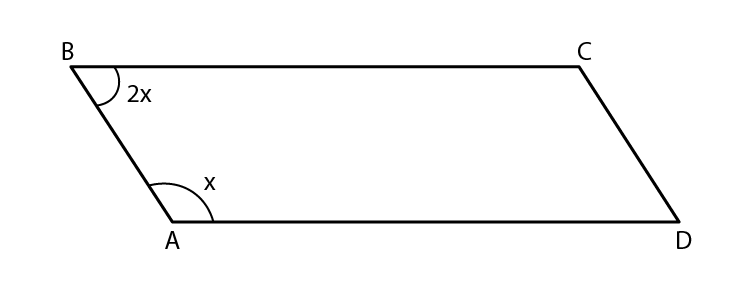Let ABCD be the parallelogram.

Given the ratio of measures of two adjacent angles is 1:2.

Let A = x

B = 2x

Adjacent angles of a parallelogram are supplementary.

A+B = 180˚

x+2x = 180

3x = 180

x = 180/3 = 60

A = 60˚

Opposite angles of a parallelogram are congruent.

C = 60˚

B = D = 2x

B = D = 2×60 = 120˚

Hence the angles of the parallelogram are 60˚,120˚,60˚ and 120˚.

5* . Diagonals of a parallelogram intersect each other at point O. If AO = 5, BO = 12 and AB = 13 then show that ABCD is a rhombus.

Solution: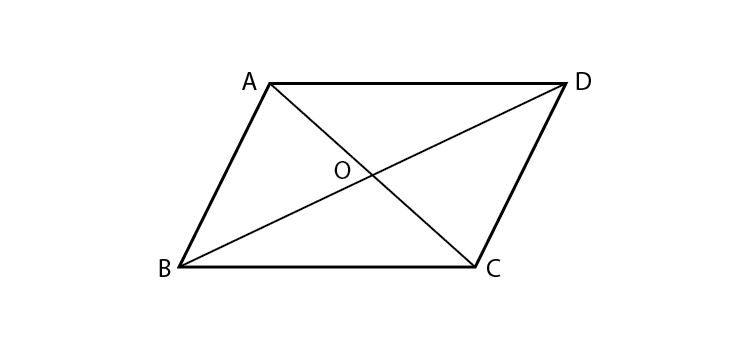Given : AO = 5, BO = 12, AB = 13

To prove : ABCD is a rhombus.

Proof:

Given AO = 5, BO = 12, AB = 13

AO2+BO2 = 52+122 = 25+144 = 169

AB2 = 132 = 169

AO2+BO2 = AB2

Hence these form a Pythagorean triplet.

AOB is a right angled triangle.

AOB = 90˚

seg AC seg BD

Hence in parallelogram ABCD, seg AC seg BD.

i.e, the diagonals are perpendicular to each other.

Rhombus is a parallelogram whose diagonals meet at right angles.

Hence ABCD is a rhombus.

Hence proved.

6. In the figure 5.12, PQRS and ABCR are two parallelograms. If P = 110° then find the measures of all angles of ABCR.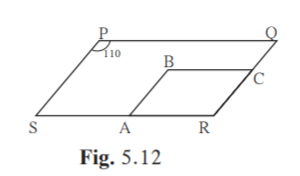Solution:

In PQRS Given P = 110˚

R = P [Opposite angles of a parallelogram are equal]

R = 110˚

In ABCR , R = 110˚

B = R [Opposite angles of a parallelogram are equal]

B = 110˚

A+B = 180˚ [Adjacent angles of a parallelogram are supplementary]

A+110˚ = 180˚

A = 180-110 = 70˚

C = A [Opposite angles of a parallelogram are equal]

C = 70˚

Hence, in ABCR, A is 70˚, B is 110˚, C is 70˚ and R is 110˚.

7. In figure 5.13 ABCD is a parallelogram. Point E is on the ray AB such that BE = AB then prove that line ED bisects seg BC at point F.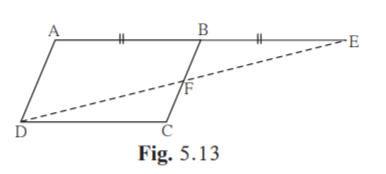Solution:

Given : E is on the ray AB such that BE = AB.

To prove: Line ED bisects seg BC at point F.

Proof:

AB CD …(i) [Opposite sides of a parallelogram are congruent]

AEDC, DE is the transversal.

BEF FDC [Alternate interior angles]

AEDC, BC is the transversal.

EBF = FCD [Alternate interior angles]

Given BE = AB

BE CD [From (i)]

By ASA test, BEF CDF.

FB FC [c.s.c.t]

Line ED bisects seg BC at point F.

Hence proved.

Practice Set 5.2 Page 67

1. In figure 5.22, ABCD is a parallelogram, P and Q are midpoints of side AB and DC respectively, then prove APCQ is a parallelogram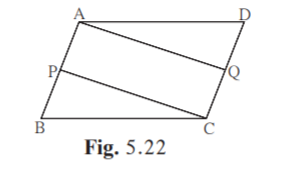Solution:

Proof:

AP = ½ AB [P is the midpoint of AB]

QC = ½ DC [Q is the midpoint of DC]

Given ABCD is a parallelogram

So AB = DC [Opposite sides of a parallelogram are equal]

½ AB = ½ DC

AP = QC…(iii) [from (i) and (ii)]

ABDC [Opposite sides of parallelogram are parallel]

APQC….(iv)

APCQ is a parallelogram. [From (iii) and (iv)]

Hence proved.

2. Using opposite angles test for parallelogram, prove that every rectangle is a parallelogram.

Solution: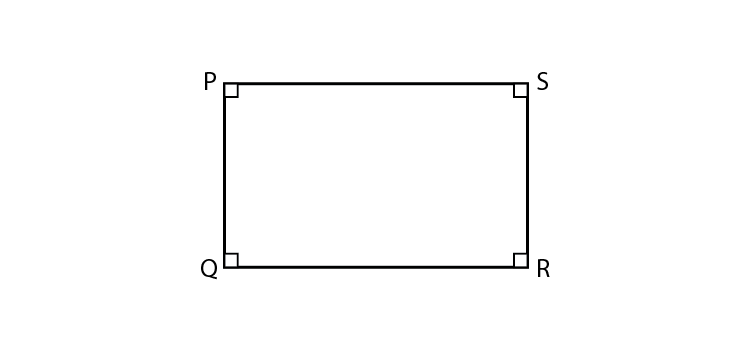Proof:

PQRS is a rectangle.

P = R = 90˚

Q = S = 90˚

Here opposite angles of the quadrilateral are congruent.

A quadrilateral is a parallelogram if its pairs of opposite angles are congruent.

Hence rectangle PQRS is a parallelogram.

Hence proved.

3. In figure 5.23, G is the point of concurrence of medians of DEF. Take point H on ray DG such that D-G-H and DG = GH, then prove that GEHF is a parallelogram.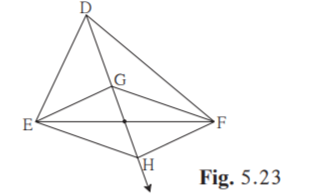Solution: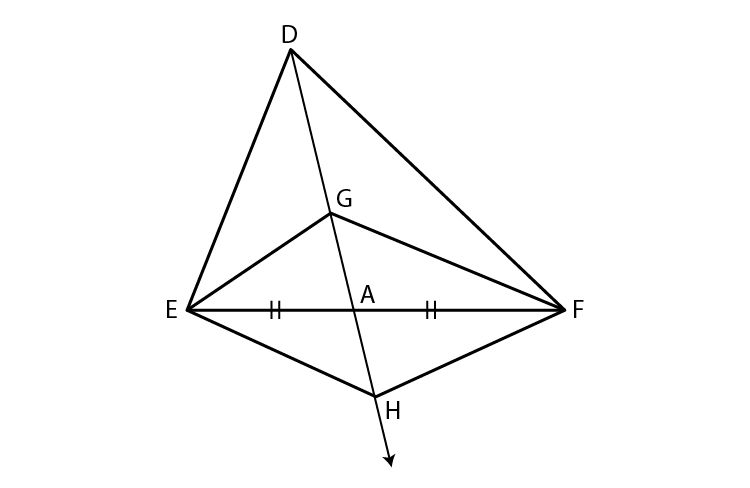Let ray DH intersect seg EF at point A such that E-A-F.

seg DA is the median of DEF.

EA = FA ……(i)

Point G is the centroid of DEF.

Since, the centroid divides each median in the ratio 2:1

DG/GA = 2/1

DG = 2(GA)

GH = 2(GA) [Given DG = GH]

GA+HA = 2(GA) [G-A-H]

HA = 2(GA) – GA

HA = GA ….(ii)

A quadrilateral is a parallelogram, if its diagonals bisect each other.

From (i) and (ii),

GEHF is a parallelogram.

Hence proved.

4. Prove that quadrilateral formed by the intersection of angle bisectors of all angles of a parallelogram is a rectangle. (Figure 5.24)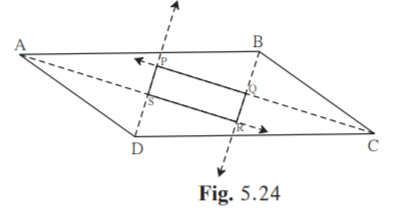Solution:

Given ABCD is a parallelogram.

DCAB. DA is the transversal.

A+D = 180˚ [Corresponding angles on same side of transversal are supplementary]

Multiply both sides by ½

½ A+ ½ D = 90˚….(i)

Let ½ A = e and ½ D = f

½ A = SAD = RAB = e [∵AS is the angle bisector of A.]

½ D = SDA = SDC = f [∵DS is the angle bisector of D.]

e+f = 90

ASD = 90˚ [Sum of all angles in ASD is 180˚]

PSR = ASD

PSR = 90˚ [Vertically opposite angles]

A+B = 180˚ [Corresponding angles on same side of transversal are supplementary]

Multiply both sides by ½

½ A+ ½ B = 90˚….(ii)

Let ½ B = g

½ A = SAD = RAB = e [∵AS is the angle bisector of A.]

½ B = ABR = CBR = g [∵BR is the angle bisector of B.]

i.e, e+g = 90˚

In ARB,

ARB+RAB+ABR = 180˚

ARB = SRQ

SRQ+e+g = 180

SRQ = 90˚ [∵e+g = 90]

DCAB. BC is the transversal.

B+C = 180˚ [Corresponding angles on same side of transversal are supplementary]

Multiply both sides by ½

½ B+ ½ C = 90˚….(ii)

½ B = QBC = ABR [∵BQ is the angle bisector of B.]

½ C = QCB [∵CQ is the angle bisector of C.]

QBC+QCB = 90˚ [From (ii)]

BQC = 90˚ [Sum of all angles in BQC is 180˚]

PQR = BQC = 90˚ [Vertically opposite angles]

PQR = 90˚

Similarly, we can prove that QPS = 90˚

Here QRS = PRS = SRQ = QPS = 90˚

Hence PQRS is a quadrilateral whose angles are 90˚ each.

PQRS is a rectangle.

Hence proved.

5. In figure 5.25, if points P, Q, R, S are on the sides of parallelogram such that AP = BQ = CR = DS then prove that PQRS is a parallelogram.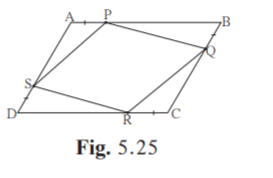Solution:

Given : ABCD is a parallelogram.

AP= BQ = CR = DS

To prove : PQRS is a parallelogram.

Proof:

AB = DC [Opposite sides of a parallelogram are equal]

AP+BP = DR+CR

AP+BP = DR+AP [Given AP = CR]

BP = DR …(i)

B = D …(ii) [Opposite angles of a parallelogram are equal]

In BPQ and DRS,

BP DR [from (i)]

BQ DS [Given]

B D [from (ii)]

By SAS test BPQ DRS.

SR = PQ …(iii) [c.s.c.t]

Similarly we can prove that PAS RCQ.

PS = RQ …(iv)

From (iii) and (iv)

PQRS is a parallelogram [If opposite sides of a quadrilateral are equal, then it is a parallelogram]

Hence proved.

Practice Set 5.3 Page 69

1. Diagonals of a rectangle ABCD intersect at point O. If AC = 8 cm then find BO and if CAD = 35° then find ACB.

Solution: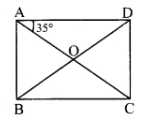Given AC = 8cm

Diagonals of a rectangle are congruent.

AC = BD

BD = 8cm

BO = ½ BD [Diagonals of a rectangle bisect each other]

BO = ½ ×8 = 4 cm

CAD = ACB [Alternate interior angles]

ACB = 35˚

Hence measure of BO is 4 cm and ACB is 35˚.

2. In a rhombus PQRS if PQ = 7.5 then find QR. If QPS = 75° then find the measure of PQR and SRQ.

Solution: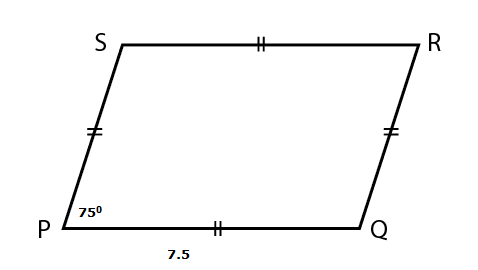Given PQ = 7.5

QR = PQ = 7.5 [All sides of rhombus are equal]

QPS = 75˚

SRQ = QPS [Opposite angles of a rhombus are equal]

SRQ = 75˚

QPS+PQR = 180˚ [Adjacent angles of a rhombus are supplementary]

75˚+PQR = 180˚

PQR = 180-75 = 105˚

Hence the measure of PQR and SRQ are 105˚ and 75˚ respectively.

3. Diagonals of a square IJKL intersects at point M, Find the measures of ∠IMJ, ∠JIK and ∠LJK .

Solution:Diagonals of a square are perpendicular bisectors of each other.

seg JL seg IK

IMJ = 90˚

JIL = 90˚ [Angle of a square]

Diagonals of a square bisect opposite angles.

JIK = 90/2 = 45˚

IJK = 90˚ [Angle of a square]

LJK = 90/2 = 45˚

Hence measures of IMJ, JIK, LJK are 90˚, 45˚ and 45˚.

4. Diagonals of a rhombus are 20 cm and 21 cm respectively, then find the side of rhombus and its perimeter.

Solution: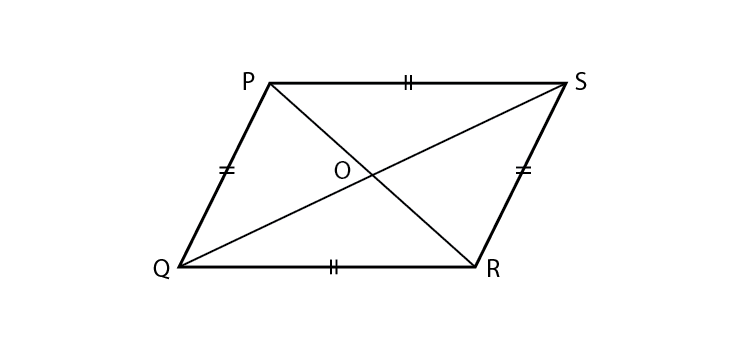Let PQRS be the rhombus.

Let PR = 20 cm

QS = 21 cm

PO = PR/2 [Diagonals of a rhombus bisect each other]

PO = 20/2 = 10 cm

QO = QS/2 [Diagonals of a rhombus bisect each other]

QO = 21/2 = 10.5 cm

POQ = 90˚ [Diagonals of a rhombus are perpendicular bisectors of each other]

In POQ ,

PQ2 = PO2+QO2 [Pythagoras theorem]

PQ2 = (20/2)2+(21/2)2

PQ = √[(400+441)/4]

PQ = √(841/4)

PQ = 29/2 = 14.5 cm

Perimeter = 4×PQ = 4×14.5 = 58 cm

Hence side and perimeter of the rhombus are 14.5 cm and 58 cm respectively.

5. State with reasons whether the following statements are ‘true’ or ‘false’.

(i) Every parallelogram is a rhombus.

(ii) Every rhombus is a rectangle.

(iii) Every rectangle is a parallelogram.

(iv) Every square is a rectangle.

(v) Every square is a rhombus.

(vi) Every parallelogram is a rectangle.

Solution:

(i) All the sides of a rhombus are congruent, while the opposite sides of a parallelogram are congruent.

Hence the statement is false.

(ii) All the angles of a rectangle are congruent, while the opposite angles of a rhombus are congruent.

Hence the statement is false.

(iii) The opposite sides of a rectangle are parallel and congruent. All its angles are congruent.

The opposite sides of a parallelogram are parallel and congruent. Its opposite angles are congruent.

Hence the statement is true.

(iv) All the sides of a square are parallel and congruent. Also, all its angles are congruent.

The opposite sides of a rectangle are parallel and congruent. Also, all its angles are congruent.

Hence the statement is true.

(v) All the sides of a square are congruent. Also, its diagonals are perpendicular bisectors of each other.

All the sides of a rhombus are congruent. Also, its diagonals are perpendicular bisectors of each other.

Hence the statement is true.

(vi) All the angles of a rectangle are congruent, while the opposite angles of a parallelogram are congruent.

Hence the statement is false.

Practice Set 5.4 Page 71

1. In IJKL, side IJ || side KL I = 108° K = 53° then find the measures of J and L.

Solution: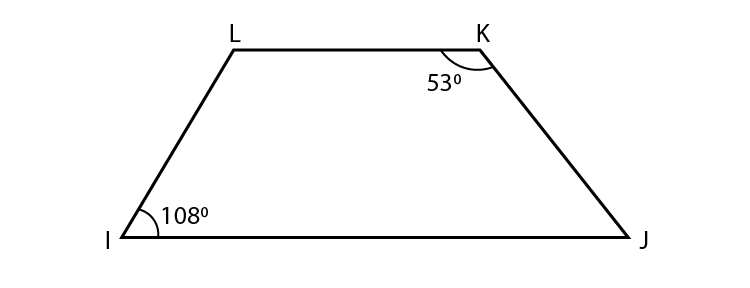Given IJKL.

IL is the transversal.

I = 108˚ [Given]

I+L = 180˚ [Interior angles on same side of transversal are supplementary]

108˚+L = 180˚

L = 180-108 = 72˚

Given IJKL.

KJ is the transversal.

K = 53˚ [Given]

K+J = 180˚ [Interior angles on same side of transversal are supplementary]

53+J = 180˚

J = 180-53 = 127˚

Hence measure of J and L are 127˚ and 72˚ respectively.

2. In ABCD, side BC || side AD, side AB side DC If A = 72° then find the measures of B, and D.

Solution:

Construction: Draw seg CQ ⊥ side AD.

Draw seg BP ⊥ side AD.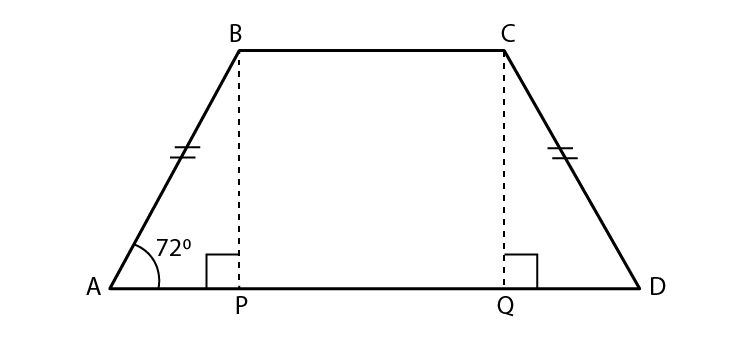A = 72° [Given]

In ABCD, side AD side BC [Given]

AB is their transversal.

A +B = 180° [Interior angles on same side of transversal]

72°+B = 180°

B = 180°–72°

B = 108°

In APB and DQC,

AB ≅ DC [Given]

APB DQC [Each angle is of measure 90°]

seg BP seg CQ [Perpendicular distance between two parallel lines]

By hypotenuse side test

APB ≅ DQC

A = D [c. a. c. t.]

D = 72°

Hence measure of B and D are 108° and 72° respectively.

3. In ABCD, side BC < side AD (Figure 5.32) side BC || side AD and if side BA side CD then prove that ABC DCB.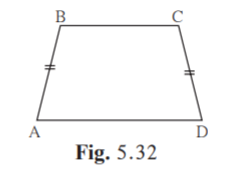Solution:

Given: side BC < side AD

side BA = side CD

To prove: ABC ≅ DCB

Construction: Draw seg BP ⊥ side AD, A-P-D

seg CQ ⊥ side AD, A-Q-D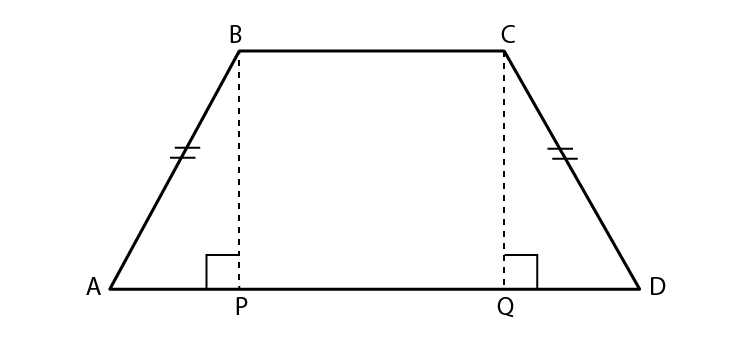Proof:

In BPA and CQD,

BPA CQB [Each angle is of measure 90°]

BA ≅ CD [Given]

seg BP seg CQ [Perpendicular distance between two parallel lines]

By Hypotenuse side test

BPA CQD

BAP CDQ [c. a. c. t.]

A = D ….(i)

side AB is their transversal. [Given]

A+B = 180°…..(ii) [Interior angles on same side of transversal]

side CD is their transversal.

C+D = 180° …..(iii) [Interior angles on same side of transversal]

Equating (ii) and (iii)

A+B = C+D [From (ii) and (iii)]

A+B = C+A [From (i)]

B = C

ABC DCB

Hence proved.

Practice set 5.5 Page 73

1. In figure 5.38, points X, Y, Z are the midpoints of side AB, side BC and side AC of ABC respectively. AB = 5 cm, AC = 9 cm and BC = 11 cm. Find the length of XY, YZ, XZ.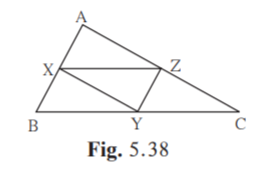Solution:

Given AC = 9 cm

X and Y are the midpoints of side AB and BC.

The segment joining midpoints of any two sides of a triangle is parallel to the third side and half of it.

XY = ½ AC

XY = ½ ×9 = 4.5 cm

Given AB = 5 cm

Z and Y are the midpoints of side AC and BC.

The segment joining midpoints of any two sides of a triangle is parallel to the third side and half of it.

YZ = ½ AB

YZ = ½ ×5 = 2.5 cm

Given BC = 11 cm

Z and X are the midpoints of side AC and AB.

The segment joining midpoints of any two sides of a triangle is parallel to the third side and half of it.

XZ = ½ BC

XZ = ½ ×11 = 5.5 cm

Hence the measures of XY, YZ and XZ are 4.5 cm, 2.5 cm and 5.5 cm.

2. In figure 5.39,PQRS and MNRL are rectangles. If point M is the midpoint of side PR then prove that, (i) SL = LR,

(ii) LN = ½ SQ.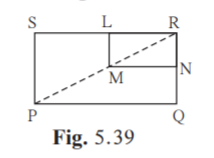Solution:

(i)Given PQRS and MNRL are rectangles.

S = 90˚ [Angle of rectangle]

L = 90˚ [Angle of rectangle]

RNLM …(i) [Opposite sides of rectangle MNRL]

RNSP ….(ii) [Opposite sides of rectangle PQRS]

From (i) and (ii)

LMSP …..(iii)

In PRS,

M is the midpoint of PR. [Given]

LMSP [From (iii)

L is the midpoint of SR …(iv) [Converse of midpoint theorem]

SL = LR

Hence proved.

(ii)Similarly for PQR we can prove that

N is the midpoint of QR …(v).

So in RSQ, N and L are the midpoints of RQ and SR respectively. [From (iv) and (v)]

LN = ½ SQ [Midpoint theorem]

Hence proved.

3. In figure 5.40, ABC is an equilateral triangle. Points F,D and E are midpoints of side AB, side BC, side AC respectively. Show that FED is an equilateral triangle.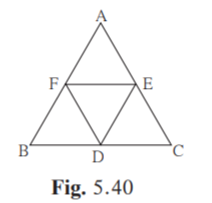Solution:

Proof:

Given ABC is an equilateral triangle.

AB = AC = BC….(i)

Points F,D and E are midpoints of side AB, side BC, side AC respectively.

D and E are the midpoints of side BC and side AC respectively.

DE = ½ AB…..(ii) [Midpoint theorem]

D and F are the midpoints of side BC and side AB respectively.

DF = ½ AC…..(iii) [Midpoint theorem]

F and E are the midpoints of side AB and side AC respectively.

FE = ½ BC…..(iv) [Midpoint theorem]

Since AB = AC = BC,

DE = DF = FE [From (ii),(iii) and (iv)]

FED is an equilateral triangle.

Hence proved.

4. In figure 5.41, seg PD is a median of PQR. Point T is the mid point of seg PD. Produced QT intersects PR at M. Show that PM/PR = 1/3 . [Hint : draw DN || QM.]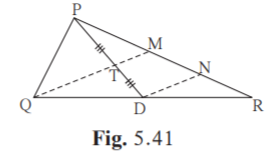Solution:

Given: PD is a median.

T is the midpoint of PD.

QT intersects PR at M

To prove :

PM/PR = 1/3

Proof:

In PDN,

Point T is the midpoint of seg PD

Given seg TM seg DN [DNQM and Q-T-M]

Point M is the midpoint of seg PN. [Construction and Q-T-M]

PM = MN ….(i) [Converse of midpoint theorem]

In QMR,

Point D is the midpoint of seg QR and seg DN seg QM [Construction]

Point N is the midpoint of seg MR. [Converse of midpoint theorem]

MN = NR …..(ii)

PM = MN = RN …..(iii) [From (i) and (ii)]

Now, PR = PM+MN+RN [ P-M-R]

PR = PM + PM + PM [From (iii) ]

PR = 3PM

PM/PR = 1/3

Hence proved.

Problem set 5 Page 73

1. Choose the correct alternative answer and fill in the blanks.

(i) If all pairs of adjacent sides of a quadrilateral are congruent then it is called ….

(A) rectangle (B) parallelogram (C) trapezium, (D) rhombus

Solution:

If all the pairs of adjacent sides of a quadrilateral are congruent, then it is a rhombus.

Hence Option D is the answer.

(ii) If the diagonal of a square is 12√2 cm then the perimeter of square is ……

(A) 24 cm (B) 24 2 cm (C) 48 cm (D) 48 2 cm

Solution: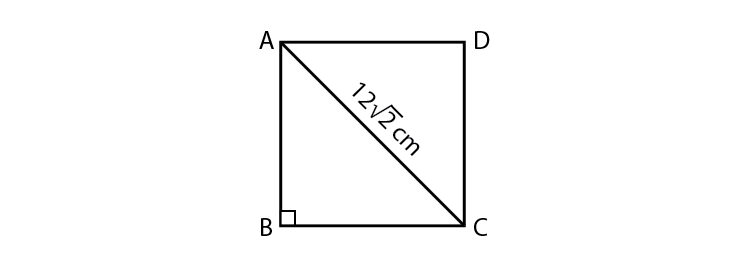Here AC = 12√2

AD = DC [sides of square are equal]

Perimeter = 4×AD = 4×12 = 48 cm

Hence Option C is the answer.

(iii) If opposite angles of a rhombus are (2x)° and (3x – 40)° then value of x is …

(A) 100 ° (B) 80 ° (C) 160 ° (D) 40

Solution:

2x = 3x-40 [Opposite angles of rhombus are equal]

2x-3x = -40

x = 40˚

Hence Option D is the answer.

2. Adjacent sides of a rectangle are 7 cm and 24 cm. Find the length of its diagonal.

Solution: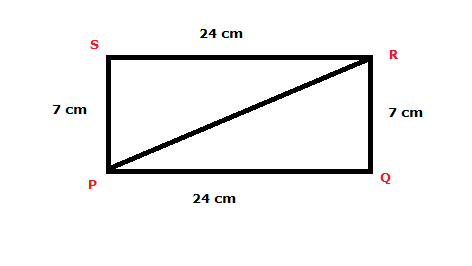Given PQ = 24 cm

QR = 7 cm

In PQR,

Q = 90˚ [Angle in a rectangle]

PR2 = PQ2+QR2 [Pythagoras theorem]

PR2 = 242+72

PR2 = 576+49

PR2 = 625

Taking square root on both sides,

PR = 25

Hence the diagonal is 25 cm long.

3. If diagonal of a square is 13 cm then find its side.

Solution: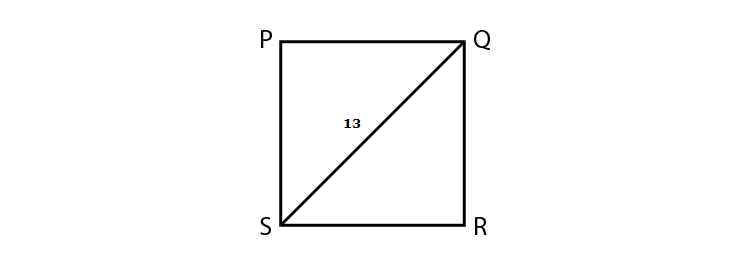Given SQ = 13 cm

R = 90˚ [Each angle of a square is 90˚]

SR = QR [All sides of a square are equal]

In SRQ ,

SQ2 = SR2+QR2

SQ2 = SR2+SR2 [SR = QR]

SQ2 = 2SR2

Taking square root on both sides

SQ = SR×√2

13 = SR×√2

SR = 13/√2 = 13×√2/(√2×√2)

= 13√2/2 = 6.5√2 cm

Hence length of side is 6.5√2 cm.

4. Ratio of two adjacent sides of a parallelogram is 3 : 4, and its perimeter is 112 cm. Find the length of its each side.

Solution: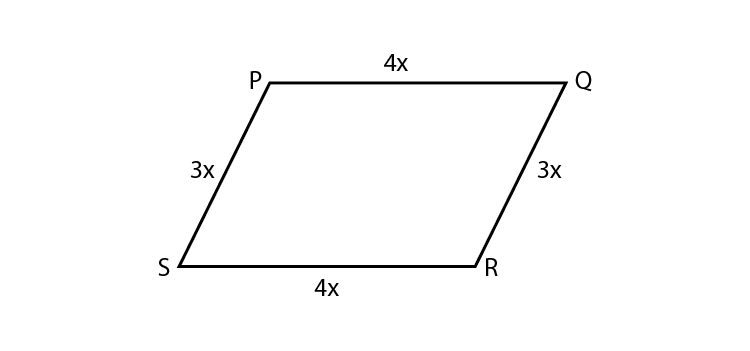Given ratio of adjacent sides of a parallelogram is 3:4.

Let PQ = SR = 4x

PS = QR = 3x

Perimeter = 112 [Given]

4x+3x+4x+3x+ = 112

14x = 112

x = 112/14 = 8

PQ = SR = 4x

PQ = SR = 4×8 = 32 cm

PS = QR = 3x

PS = QR = 3×8 = 24 cm

Hence lengths of the sides of the parallelogram are 32 cm, 24 cm , 32 cm and 24 cm .

5. Diagonals PR and QS of a rhombus PQRS are 20 cm and 48 cm respectively. Find the length of side PQ.

Solution: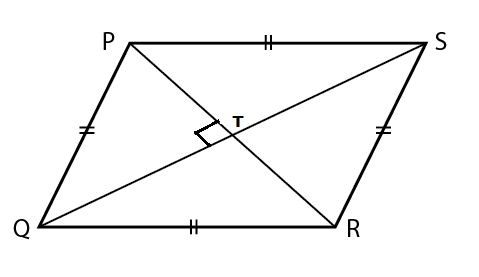Given PR = 20

QS = 48

QT = QS/2 = 48/2 = 24 [Diagonals of a rhombus bisect each other]

PT = PR/2 = 20/2 = 10 [Diagonals of a rhombus bisect each other]

In PQT,

PTQ = 90˚ [Diagonals of a rhombus are perpendicular bisectors of each other.]

PQ2 = PT2+QT2

PQ2 = 102+242

PQ2 = 100+576

PQ2 = 676

Taking square root on both sides,

PQ = 26

Hence measure of side PQ is 26 cm.

6. Diagonals of a rectangle PQRS are intersecting in point M. If QMR = 50° then find the measure of MPS.

Solution: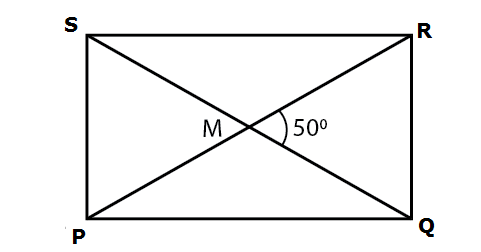Given QMR = 50˚

SMP = 50˚ [Vertically opposite angles]

PR = SQ …(i) [Diagonals of a rectangle are congruent]

PM = ½ PR …(ii) [Diagonals of a rectangle bisect each other]

MS = ½ SQ….(iii) [Diagonals of a rectangle bisect each other]

PM = MS…(iv) [From (i) ,(ii) and (iii)]

MSP = MPS…(v) [Isoceles triangle theorem]

In SMP,

MSP +MPS+SMP = 180˚ [Angle sum property of triangle]

MPS+MPS+50˚ = 180˚ [From (i) and (v)]

2MPS = 180-50 = 130˚

MPS = 130/2 = 65˚

Hence measure of MPS is 65˚.

7. In the adjacent Figure 5.42, if seg AB || seg PQ , seg AB @ seg PQ, seg AC || seg PR, seg AC seg PR then prove that, seg BC || seg QR and seg BC seg QR.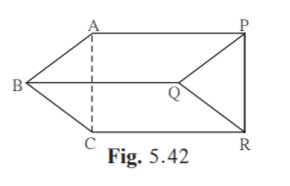Solution:

Given: seg AB seg PQ ,

seg AB seg PQ

seg AC seg PR

seg AC seg PR

To prove: seg BC seg QR and seg BC seg QR

Proof:

In ABQP

seg AB seg PQ [Given]

seg AB seg PQ [Given]

ABQP is a parallelogram. [A quadrilateral is a parallelogram if a pair of its opposite sides is parallel and congruent]

segAP segBQ …..(i)

seg AP seg BQ …..(ii) [Opposite sides of a parallelogram are congruent]

In ACRP,

seg AC seg PR [Given]

seg AC seg PR [Given]

ACRP is a parallelogram. [A quadrilateral is a parallelogram if a pair of its opposite sides is parallel and congruent]

seg AP seg CR …(iii)

seg AP seg CR …….(iv) [Opposite sides of a parallelogram are congruent]

In BCRQ,

seg BQ seg CR

seg BQ seg CR

BCRQ is a parallelogram. [A quadrilateral is a parallelogram if a pair of its opposite sides is parallel and congruent]

seg BC seg QR

seg BC seg QR [Opposite sides of a parallelogram are congruent]

Hence Proved.

8* . In the Figure 5.43,ABCD is a trapezium. AB DC. Points P and Q are midpoints of seg AD and seg BC respectively. Then prove that, PQ || AB and PQ = ½ (AB + DC)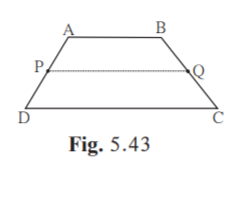Solution: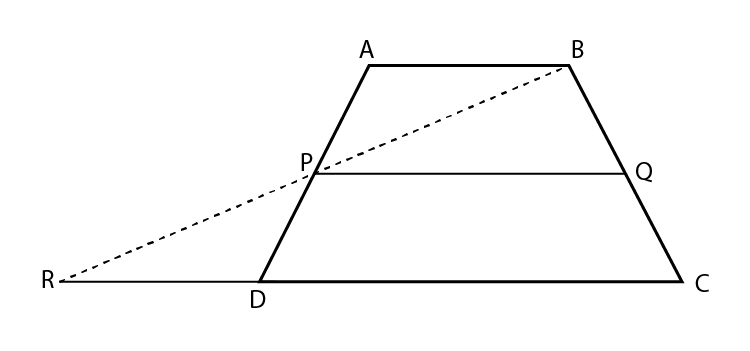Construction: Join BP and extend it to meet CD produced at R.

To prove: PQ AB and  PQ = ½ (AB+DC)

Proof:

In ABP and DRP,

APB =DPR              [Vertically opposite angles]

PDA =PAB              [Alternate interior angles ]

AP = PD                            [P is the mid point of DA]

APB = DPR             [Vertically opposite angles]

PDA = PAB             [ Alternate interior angles]

AP = PD                            [P is the mid point of DA]

By ASA test  ABP  DRP.

PB = PR [c.s.c.t]

AB = RD [c.s.c.t]

In BRC,

Given Q is the mid point of BC

P is the mid point of BR                      [ ∵PB = PR]

So, by midpoint theorem, PQ RC

⇒⇒PQ DC

Given AB DC

So, PQ AB.

Also, PQ = ½ RC

PQ = ½ (RD+DC)

PQ = ½ (AB+DC)  [∵AB = RD]

Hence proved.

9. In the adjacent figure 5.44, �ABCD is a trapezium. AB || DC. Points M and N are midpoints of diagonal AC and DB respectively then prove that MN || AB.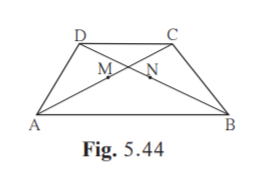Solution:

Given: ABCD is a trapezium.

AB DC.

Points M and N are midpoints of diagonals AC and DB respectively.

To prove: MN AB

Construction: Join D and M. Extend seg DM to meet seg AB at point E such that A-E-B.

Proof:

Given seg AB seg DC

seg AC is their transversal.

CAB ACD [Alternate interior angles]

MAE MCD ….(i) [C-M-A, A-E-B]

In AME and CMD,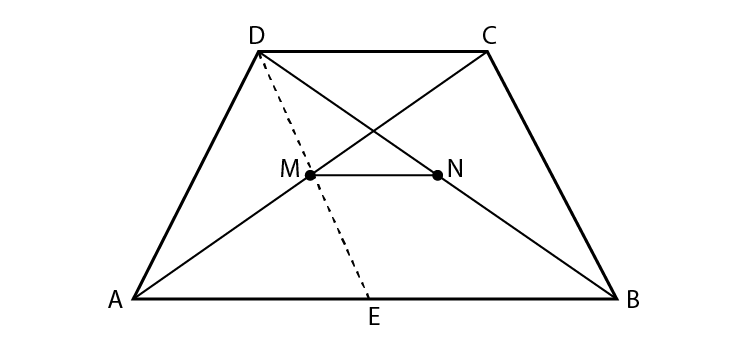AME CMD [Vertically opposite angles]

seg AM seg CM [M is the midpoint of AC]

MAE MCD [From (i)]

By ASA test AME CMD

seg ME seg MD [c.s.c.t]

Point M is the midpoint of seg DE. …(ii)

In DEB,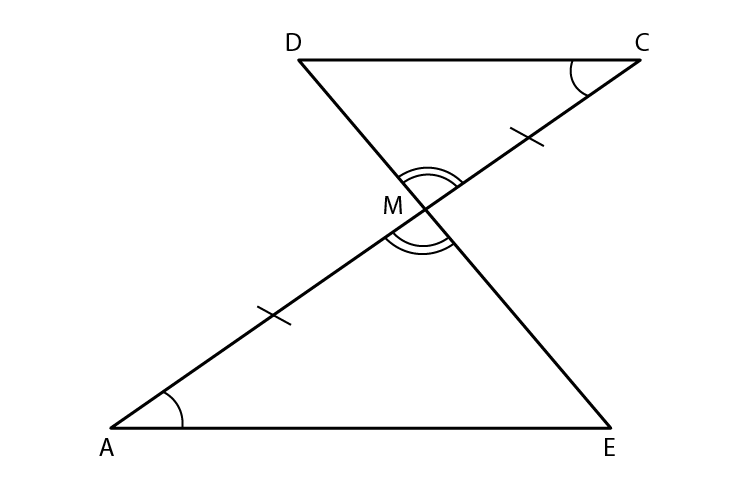Given points M and N are the midpoints of DE and DB respectively.

seg MN seg EB [Midpoint theorem]

seg MN seg AB [A-E-B]

Hence proved.

This chapter deals with the different tests of a parallelogram. Midpoint theorem is also explained in this chapter. These solutions help the students to make their learning easy and improve their confidence. Get thorough with the concepts of Quadrilaterals and various problems with BYJU’S.

## Frequently Asked Questions on Maharashtra State Board Solutions for Class 9  Maths Part 2 Chapter 5 Quadrilaterals

### Can we use these Maharashtra State Board Class 9 Maths Part 2 Chapter 5 Solutions to prepare for the exams?

Yes, these solutions will help to prepare for the board exams. Students are highly recommended to practise these solutions after revising the subjects, as they set the basis for the questions that could get asked in the board exams.

### How can students make the best use of these solutions?

Students are encouraged to practise these questions at first. Then they can refer back to the solutions to analyse their performance. This will also help the students to rectify the mistakes and avoid making them during the board exams.

### Is is possible to download these Maharashtra State Board Class 9 Maths Part 2 Chapter 5 Solutions?

If a sufficiently large quantity of solvent dissolves a small volume of solvent, the solution is considered a dilute solution. Concentrated solution is when the quantity of solvent dissolved is relatively high compared to the quantity of water, then concentrated solution is called solution.